# EP1780675A1 - Object detector - Google Patents

## Info

Publication number
EP1780675A1
EP1780675A1 EP05770610A EP05770610A EP1780675A1 EP 1780675 A1 EP1780675 A1 EP 1780675A1 EP 05770610 A EP05770610 A EP 05770610A EP 05770610 A EP05770610 A EP 05770610A EP 1780675 A1 EP1780675 A1 EP 1780675A1
Authority
EP
European Patent Office
Prior art keywords
object
image
position
unit
calculating
Prior art date
Legal status (The legal status is an assumption and is not a legal conclusion. Google has not performed a legal analysis and makes no representation as to the accuracy of the status listed.)
Granted
Application number
EP05770610A
Other languages
German (de)
French (fr)
Other versions
EP1780675A4 (en
EP1780675B1 (en
Inventor
Akihito c/o Tokyo Institute of Technology SEKI
Masatoshi c/o Tokyo Inst. of Technology OKUTOMI
Tomo c/o K. K. Honda Gijutsu Kenkyusho FUJIMAKI
Current Assignee (The listed assignees may be inaccurate. Google has not performed a legal analysis and makes no representation or warranty as to the accuracy of the list.)
Honda Motor Co Ltd
Tokyo Institute of Technology NUC
Original Assignee
Honda Motor Co Ltd
Tokyo Institute of Technology NUC
Priority date (The priority date is an assumption and is not a legal conclusion. Google has not performed a legal analysis and makes no representation as to the accuracy of the date listed.)
Filing date
Publication date
Priority to JP2004234947A priority Critical patent/JP4328692B2/en
Application filed by Honda Motor Co Ltd, Tokyo Institute of Technology NUC filed Critical Honda Motor Co Ltd
Priority to PCT/JP2005/014785 priority patent/WO2006016663A1/en
Publication of EP1780675A1 publication Critical patent/EP1780675A1/en
Publication of EP1780675A4 publication Critical patent/EP1780675A4/en
Application granted granted Critical
Publication of EP1780675B1 publication Critical patent/EP1780675B1/en
Application status is Expired - Fee Related legal-status Critical
Anticipated expiration legal-status Critical

## Images

•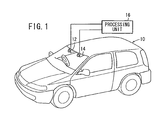•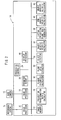•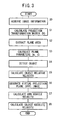•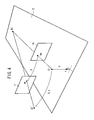••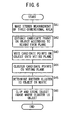••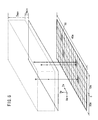•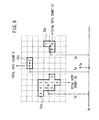•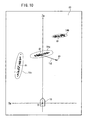•••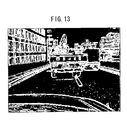••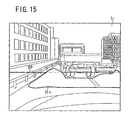•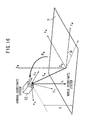•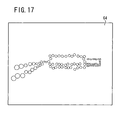•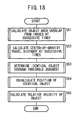•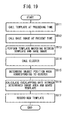••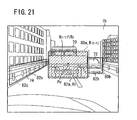•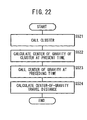•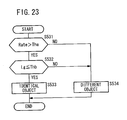••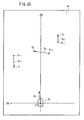•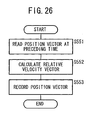•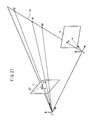•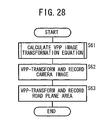•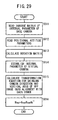•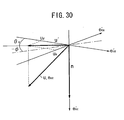•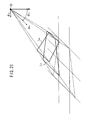•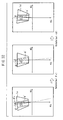•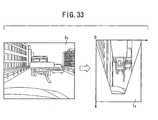•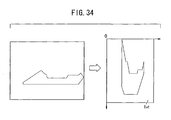•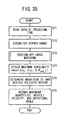•••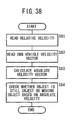•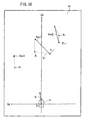## Classifications

• GPHYSICS
• G06COMPUTING; CALCULATING; COUNTING
• G06KRECOGNITION OF DATA; PRESENTATION OF DATA; RECORD CARRIERS; HANDLING RECORD CARRIERS
• G06K9/00Methods or arrangements for reading or recognising printed or written characters or for recognising patterns, e.g. fingerprints
• G06K9/00624Recognising scenes, i.e. recognition of a whole field of perception; recognising scene-specific objects
• G06K9/00791Recognising scenes perceived from the perspective of a land vehicle, e.g. recognising lanes, obstacles or traffic signs on road scenes
• G06K9/00805Detecting potential obstacles
• GPHYSICS
• G06COMPUTING; CALCULATING; COUNTING
• G06TIMAGE DATA PROCESSING OR GENERATION, IN GENERAL
• G06T7/00Image analysis
• G06T7/70Determining position or orientation of objects or cameras
• G06T7/73Determining position or orientation of objects or cameras using feature-based methods
• G06T7/74Determining position or orientation of objects or cameras using feature-based methods involving reference images or patches
• GPHYSICS
• G06COMPUTING; CALCULATING; COUNTING
• G06KRECOGNITION OF DATA; PRESENTATION OF DATA; RECORD CARRIERS; HANDLING RECORD CARRIERS
• G06K9/00Methods or arrangements for reading or recognising printed or written characters or for recognising patterns, e.g. fingerprints
• G06K9/20Image acquisition
• G06K9/32Aligning or centering of the image pick-up or image-field
• G06K2009/3291Pattern tracking

## Abstract

An object position area (82a) is calculated according to a position in the space of a detected truck (70). A template (80a) corresponding to the object position area (82a) recorded at the previous time is then called. The template (80a) is moved to a position on a reference image Ib where the similarity is highest. Overlap rate (R(t-1)∩Rt) of the object position area (82a) and the template (80a) is calculated. A decision is made whether the object is identical to that detected in the past by using the overlap rate.

## Description

TECHNICAL FIELD
• The present invention relates to an object detecting apparatus for detecting a peripheral object based on an acquired image.
• BACKGROUND ART
• Processes for detecting a road plane area that can be traveled or detecting an obstacle that is present in a traveling environment in guiding a mobile object such as an automobile or the like are roughly classified into processes using a laser radar, ultrasonic waves, a milliwave radar, etc., and processes using images.
• The detecting processes using a laser radar and a milliwave radar are problematic in that the apparatus used are generally expensive and a sufficient spatial resolution cannot be achieved. The detecting processes using ultrasonic waves are problematic in that it is difficult to make measurements in distant ranges and the spatial resolution is low.
• The processes using images are grouped into single-eyed processes and double-eyed processes. Heretofore, many of the processes using images employ an image obtained from a single eye, i.e., a single viewpoint. They are assumed to be used in a well-maintained environment such as an expressway or the like, and detect a traveling area by detecting a white line on the road (for example, a separation line or a center line). However, in general roads and parking lots where the presence of white lines or the like is not guaranteed and various road plane colors and patterns are present, it is difficult to stably distinguish between areas that can be traveled and obstacles, only from a density pattern obtained from a single-eyed image.
• The double-eyed processes, i.e., processes using a stereo image, make it possible to recognize a traveling environment more stably because they can make use of a three-dimensional structure of the traveling environment in principle. Particularly, since an area that can be traveled can be regarded essentially as a plane in space, there has been proposed a process of calculating a projection transformation matrix between images, transforming one of the images into a two-dimensional projection image according to the projection transformation matrix, overlapping the transformed image onto the other image, and detecting whether the image represents a plane area or not or an obstacle or not, based on the overlapping (see Patent Document 1, Non-patent Document 1).
• Patent Document 2 discloses an obstacle detecting apparatus. In order to estimate an object area in an image, the obstacle detecting apparatus determines edges of the object area from density change in the image and estimates the object area from an image area with motion using an optical flow detecting means. Then, the apparatus checks matching of position and size in the image with respect to an object area detected by a feature extracting means and an object area detected by the optical flow detecting means, thereby detecting an identical object.
• Patent Document 1: Laid-Open Patent Publication 2000-293693 (Japan );
• Patent Document 2: Laid-Open Patent Publication 2001-21345 (Japan ); and
• Non-patent Document 1: Heung-Yeung Shum, Richard Szeliski, "Panoramic Image Mosaics" Technical report, 1997, MSR-TR-97-23, Microsoft Research.
DISCLOSURE OF THE INVENTION
• When a vehicle with an object detecting apparatus travels outside, object edges are not necessarily stably determined due to effects of shadows or the like resulting from brightness changes depending on the weather and time. Thus, it is difficult to estimate an object area.
• In the image of a complicated scene, there are often some background objects overlapping, other than objects on the road plane. Therefore, when edges are to be extracted from such an image based on density changes, it is likely to estimate an object as including the background objects.
• Further, since the image captured by a camera is two-dimensional information, depth information of an object photographed is lost. Because motion of an object in space is observed in the image, as the motion on a two-dimensional plane, a plurality of objects spaced away from each other in space is observed in the image, as the objects being in proximity to or overlapping each other. Therefore, when motion of a plurality of objects is to be observed in the image using an optical flow or the like, the motion of the objects often cannot be traced due to effects of the proximity or the overlapping in the image.
• For example, when a periphery of a vehicle traveling a general road, such as the front or the back, is captured by an in-vehicle camera from the vehicle, there are some moving objects, background objects, etc. captured in the image. In the image captured at each time in the above situation, proximity or overlapping in the image frequently occurs depending on the positional relationship of the objects in space.
• Also, when a plurality of objects in an image are observed as being in proximity to each other, the obstacle detecting apparatus according to Patent Document 2 may estimate an area enclosing an edge set of the objects as one object area. When a template corresponding to the object area is generated based on the above result, and correlation operation is performed on the images at successive times, accurate detecting process cannot be performed because of low correlation if the objects are moving away from each other in the image.
• It is a main object of the present invention to provide an object detecting apparatus which can identify a plurality of objects individually and stably even if the objects are in proximity to or partially overlap each other in an image.
• Another object of the present invention is to trace an object more reliably, in view of travel distance with respect to a center-of-gravity position.
• Further object of the present invention is to calculate a relative velocity highly accurately with variation on measure points reduced and to improve accuracy in stereo measurement.
• With an object detecting apparatus according to the present invention, since object tracing is performed using the correlation of features in an image and the positional relationship of features in space, even if near objects and remote objects are observed in the image as being in proximity to or partially overlapping each other, the apparatus can distinguish between the near objects and the remote objects and associate them with each other, respectively. Therefore, it is possible to accurately associate an object position area at each time with an object and to stably identify each object. Also, in comparison with the object tracing using only positional relationship in space, it is possible to trace objects more stably when correlation on the image is considered.
• Furthermore, it is possible to trace an object more reliably in view of travel distance with respect to a center-of-gravity position.
• With the object detecting apparatus installed in a vehicle, the relative velocity of an object can be determine accurately and the absolute velocity of the detected object can be determined accurately based on a mobile object's own velocity and the relative velocity of the detected object. Thus, it can be judged accurately whether the detected object is a still object or not.
• BRIEF DESCRIPTION OF THE DRAWINGS
• FIG. 1 is a view illustrative of a vehicle to which an object detecting apparatus according to the present invention is applied;
• FIG. 2 is a functional block diagram of a processing unit mounted on the vehicle shown in FIG. 1;
• FIG. 3 is a flowchart of an overall processing sequence of the processing unit shown in FIG. 1;
• FIG. 4 is a diagram illustrative of a two-dimensional projection transformation between a pair of image capturing means;
• FIG. 5 is a diagram illustrative of a process of extracting a plane area;
• FIG. 6 is a flowchart of a processing sequence of an object extracting process;
• FIG. 7 is a diagram showing the manner in which a candidate point is extracted based on a height limitation;
• FIG. 8 is a diagram showing the manner in which a voting process is performed;
• FIG. 9 is a diagram showing a clustering process performed on a voting plane;
• FIG. 10 is a diagram showing a voting plane;
• FIG. 11 is a flowchart of a processing sequence for determining world coordinates of each edge point of an object;
• FIG. 12 is a diagram showing an edge image of extracted edges;
• FIG. 13 is a diagram showing actual image data of the edge image of extracted edges;
• FIG. 14 is a diagram showing a corrected edge image with a plane area removed;
• FIG. 15 is a diagram showing a reference image with an epipolar line established therein;
• FIG. 16 is a diagram showing a relationship between a world coordinate system and a camera coordinate system;
• FIG. 17 is a diagram showing a depth image;
• FIG. 18 is a flowchart of a processing sequence for calculating a relative velocity of an object;
• FIG. 19 is a flowchart of a processing sequence for checking how an object area is overlapped;
• FIG. 20 is a diagram showing a process of generating a template based on a cluster;
• FIG. 21 is a diagram showing a base image with a template established therein;
• FIG. 22 is a flowchart of a processing sequence for calculating a center-of-gravity travel distance;
• FIG. 23 is a flowchart of a processing sequence for determining an object identity;
• FIG. 24 is a flowchart of a processing sequence for recalculating the position of an identical object;
• FIG. 25 is a diagram showing a voting plane in which a relative velocity of an object is recorded;
• FIG. 26 is a flowchart of a processing sequence for calculating a relative velocity of an object;
• FIG. 27 is a diagram showing a process of establishing an epipolar line;
• FIG. 28 is a flowchart showing a process of generating a VPP image;
• FIG. 29 is a flowchart showing a process of calculating a VPP image transformation formula;
• FIG. 30 is a coordinate diagram showing a relationship between a normal vector, a camera coordinate system, and the optical axis of a base camera;
• FIG. 31 is a diagram showing a process of transforming a camera coordinate system base image into an image parallel to a road plane;
• FIG. 32 is a diagram showing a rotational transformation of a first transformed image;
• FIG. 33 is a diagram showing a VPP transformation of a base image;
• FIG. 34 is a diagram showing a VPP transformation of a road plane area;
• FIG. 35 is a flowchart of a processing sequence for calculating an own vehicle velocity;
• FIG. 36 is a flowchart of a processing sequence of a VPP image matching process;
• FIG. 37 is a block diagram showing processing details of the VPP image matching process;
• FIG. 38 is a block diagram showing a processing sequence for calculating an absolute velocity of an object; and
• FIG. 39 is a diagram showing a voting plane in which an absolute velocity of an object is recorded.
BEST MODE FOR CARRYING OUT THE INVENTION
• FIG. 1 shows a vehicle 10 according to an embodiment to which an object detecting apparatus according to the present invention is applied. The vehicle 10 has a windshield on which there are fixedly mounted a base camera 12 and a reference camera 14 in upper left and right regions thereof as a pair of image capturing means for capturing images including a road plane area where the vehicle 10 travels. The base camera 12 and the reference camera 14 are stereo cameras comprising CCD cameras or the like with a common image capturing area set therein. The base camera 12 and the reference camera 14 are connected to a processing unit 16 for processing captured images to detect the road plane area and an object which may possibly become an obstacle. In the description which follows, an image captured by the base camera 12 will be referred to as a base image, and an image captured by the reference camera 14 as a reference image.
• FIG. 2 is a functional block diagram of the processing unit 16. The processing unit 16 has an image processor 18 for processing a plurality of base images captured by the base camera 12, an image processor 20 for processing a plurality of reference images captured by the reference camera 14, and an image memory 22 for storing base images and reference images that are captured at given image capturing time intervals.
• The processing unit 16 also has a processing controller 24 to which there are connected the image memory 22, a parameter memory 26, a projection transformation matrix calculator 28, a plane parameter calculator 30, a plane area extractor 32, an object detector 34, an object relative velocity calculator 36, a virtual projection plane image generator 38, an own vehicle velocity calculator 40, an object absolute velocity calculator 42, and a display processor 44.
• The parameter memory 26 stores initial value parameters required for processing operation and parameters calculated in previous and present processing cycles. The projection transformation matrix calculator 28 calculates a projection transformation matrix for performing a two-dimensional projection transformation from a road plane area in a reference image into a road plane area in a base image. The plane parameter calculator 30 calculates a normal vector to a road plane and a distance from the optical center of the base camera 12 to a road plane as plane parameters. The plane area extractor 32 extracts a road plane area that can be traveled by the vehicle 10, using a base image and a reference image projectively transformed by a projection transformation matrix. The object detector 34 detects an object that is present in an area outside of a road plane area. The object relative velocity calculator 36 calculates a relative velocity vector of an extracted object with respect to the vehicle 10. The virtual projection plane image generator 38 generates a virtual projection plane (VPP) image representative of an extracted road plane area as projected onto a virtual plane parallel to an actual road plane. The own vehicle velocity calculator 40 calculates an own vehicle velocity vector of the vehicle 10 on a VPP image. The object absolute velocity calculator 42 calculates an absolute velocity vector of an object from a relative velocity vector of the object with respect to the vehicle 10 and an own vehicle velocity vector of the vehicle 10. The display processor 44 displays the above processed results on a display, and announces a warning or the like based on the processed results.
• The vehicle 10 to which the object detecting apparatus according to the present invention is applied and the processing unit 16 are basically constructed as described above. An overall processing sequence of the processing unit 16 will be described below based on a flowchart shown in FIG. 3.
• First, the base camera 12 and the reference camera 14 captures base images and reference images including a road plane area which is being traveled by the vehicle 10, at given time intervals. After the images are processed by the image processors 18, 20, they are stored as image information in the image memory 22 at each time (step S0).
• Then, the projection transformation matrix calculator 28 dynamically calculates a projection transformation matrix for performing a two-dimensional projection transformation from a road plane area in a reference image into a road plane area in a base image, using the initial value parameters stored in the parameter memory 26 (step S1).
• The plane area extractor 32 extracts the road plane area from a base image, using the projection transformation matrix calculated in step S1 (step S2).
• The plane parameter calculator 30 calculates a normal vector to the road plane and a distance from the optical center of the base camera 12 to the road plane as plane parameters (step S3).
• Then, the object detector 34 transforms the road plane area extracted in step S2 onto a road plane, using the plane parameters calculated in step S3, and detects objects which may possibly become obstacles other than the vehicle 10, or alternatively makes a stereo measurement with respect to an area other than the road plane area extracted in step S2, and detects objects (step S4).
• The object relative velocity calculator 36 calculates a relative velocity vector of each object with respect to the vehicle 10, from positional changes at each time of the respective extracted objects (step S5).
• The virtual projection plane image generator 38 generates a virtual projection plane (VPP) image representative of a road plane area as viewed from above, using the normal vector which indicates the gradient of the road plane calculated in step S3 (step S6).
• The own vehicle velocity calculator 40 calculates an own vehicle velocity vector of the vehicle 10 from the position at each time in the VPP image generated in step S6 (step S7).
• The object absolute velocity calculator 42 calculates an absolute velocity vector of each object from the relative velocity vector of the object calculated in step S5 and the own vehicle velocity vector of the vehicle 10 calculated in step S7 (step S8).
• The display processor 44 displays the road plane area extracted as described above, the objects which may possibly become obstacles, the absolute velocities of the vehicle 10 and the objects, etc. on the display, and, if necessary, announces a warning based on the relative positional and velocity relationships between the vehicle 10 and the objects, for example.
• The present embodiment will be described in detail below with respect to each of the steps.
• First, the principles of the extraction of a road plane area and the two-dimensional projection transformation used in the present invention will be described below. In the description which follows, homogeneous coordinates will be denoted by reference characters followed by a symbol "^" in the description, and reference characters capped with a symbol "-" in the equations and the expressions. Projectively transformed images will be denoted by reference characters followed by a symbol "'"in the description, and reference characters capped with a symbol "-" in the equations and the expressions.
• As shown in FIG. 4, it is well known in the art that when an observational point M1 on a plane Π in space (also referred to as a road plane) is projected onto a base image Ib and a reference image Ir, homogeneous coordinates m^b on the base image Ib and homogeneous coordinates m^r on the reference image Ir are related to each other by a two-dimensional projection transformation as indicated by the expression (1-1): $m ˜ r ∼ H ⁢ m ˜ b$
("-" between both sides represents equality therebetween while allowing indefiniteness of a constant multiplication)
• In the above expression, H represents a 3 × 3 projection transformation matrix. In FIG. 4, O represents the optical center of the base camera 12, O' the optical center of the reference camera 14, R a rotation matrix from a base camera coordinate system to a reference camera coordinate system, t a translation vector directed from the optical center of the reference camera 14 to the optical center of the base camera 12 in the reference camera coordinate system, d the distance between the optical center O of the base camera 12 and the plane Π, and n the normal vector to the plane Π.
• If an area that can be traveled, such as a road, can be regarded as being essentially a plane in space, then it is possible to obtain an image that agrees with the base image Ib with respect to the plane by performing an appropriate projection transformation on the area with respect to the reference image Ir. On the other hand, an object which is not present on the plane produces a projectively transformed image which does not agree with the base image Ib, and thus the area of the object can be judged not as an area that can be traveled, or can be judged as an area which could become an obstacle. Therefore, an area that can be traveled or an area which can be an obstacle can be detected by calculating the expansiveness of the plane.
• <step S0>
• Image information of the base image Ib and the reference image Ir is acquired.
• Images are captured using the base camera 12 and the reference camera 14. In order to remove the brightness difference between the images, the images are subjected to LOG (Laplacian of Gaussian) filters by the image processors 18, 20. Since the images processed by the LOG filters have a low contrast level, they are subjected to histogram equalization. The base image Ib and the reference image Ir thus processed and their original images are stored in the image memory 22.
• <step S1>
• The projection transformation matrix calculator 28 dynamically calculates a projection transformation matrix H with respect to a road plane, using the base image Ib and the reference image Ir that are stored in the image memory 22.
• Specifically, within a certain area R1 in the base image Ib, the projection transformation matrix H is changed slightly, and a projection transformation matrix H for minimizing an evaluation function E(H) according to the expression (1-2) below is estimated by repetitive optimization. $E H = ∑ m b ∈ R I I r H ⁢ m ˜ b - I b m ˜ b 2$
where Ib(m^b), Ir(Hm^b) represent respective luminance values of the base image Ib and the reference image Ir at the homogeneous coordinates m^b, Hm^b.
• The above estimation requires the projection transformation matrix H as an initial value that is appropriately close to a true value and an area R1 (according to the present invention, hereinafter called "calculation area"). The projection transformation matrix H and the calculation area R1 are then determined according to a process described below, using time-series information.
• A projection transformation matrix Hs between a base image Ib and a reference image Ir at the same time, a projection transformation matrix Hm between two base images Ib acquired at successive times, and a calculation area R1 with respect to a base image Ib are determined. For estimating a projection transformation matrix H (Hs, Hm) at a certain time t, estimated results up to a preceding time (t-1) are employed.
• First, a projection transformation matrix Hm(t) between successive base images Ib(t-1) and Ib(t), is estimated by repetitive optimization based on the expression (1-2). At this time, a projection transformation matrix Hm(t-1) estimated at the preceding time may be used as an initial value of the projection transformation matrix Hm(t), and a calculation area R1(t-1) determined at the preceding time with respect to a base image Ib(t-1) may be used as an initial value of the calculation area R1.
• Then, using the determined projection transformation matrix Hm(t), the calculation area R1(t-1) at the preceding time is transformed to determine a predicted value of a calculation area R1(t) at the present time.
• A projection transformation matrix Hs(t) between a base image Ib and a reference image Ir is estimated by repetitive optimization based on the expression (1-2), using the projection transformation matrix Hs(t-1) estimated at the preceding time as an initial value and also using the calculation area R1(t) determined from the projection transformation matrix Hm(t).
• In the successive estimation using time-series images, a dynamic projection transformation matrix Hs (hereinafter referred to as a projection transformation matrix H) between a base image Ib and a reference image Ir at the time t can stably be estimated using the projection transformation matrix H as an initial value that is appropriately close to a true value and the calculation area R1.
• A process of estimating the projection transformation matrix H according to repetitive optimization (density gradient method) using the expression (1-2) will be described in detail below.
• The density gradient method is a method of estimating a change in the projection transformation matrix H for maximum image overlapping by changing one of two images slightly and minimizing an evaluation function representing how the images are overlapped (density difference).
• If a luminance value of a reference image Ir at coordinates x (x, y) is represented by Ir(x), then a reference image Ir' projectively transformed by an appropriate projection transformation matrix H is expressed by the expression (1-3) shown below. The symbol "~" above the reference image Ir indicates an image that has been projectively transformed, and the symbol "-" above x indicates homogeneous coordinates of the coordinates x (x, y). $I ˜ r x ˜ = I r H ⁢ x ˜$
• The projection transformation matrix H is a matrix with 8 degrees of freedom (one parameter being fixed) made up of 3 × 3 parameters.
• If, when the 8 parameters of the projection transformation matrix H are changed slightly, the coordinates x (x, y) on the reference image Ir' that has been projectively transformed change to coordinates x" (x", y"), then the relationship expressed by: $x ˜ ⁢ ʹʹ ∼ I + D x ⁢ x ˜$ ("~" between both sides represents equality therebetween while allowing indefiniteness of constant multiplication) is obtained. In the above expression, I represents a 3 × 3 unit matrix, and Dx a 3 × 3 slightly changed matrix having, as parameters thereof, slight changes in the parameters of the projection transformation matrix H.
• Using the relationships represented by the expressions (1-3), (1-4), the evaluation function E(H) according to the expression (1-2), which represents an overlapped state of the projectively transformed reference image Ir' and the base image Ib is expressed, using as variables, the parameters of the slightly changed matrix Dx of the projection transformation matrix H, as follows: $E d x = ∑ i I ˜ r x ˜ i ⁢ ʹʹ - I b x ˜ i 2$
("i" represents a pixel number)
In the above expression, dx represents a parameter of the slightly changed matrix Dx as expressed in terms of a vector.
• When the expression (1-5) is Taylor expanded for first-order approximation, it is expressed as follows: $E d x ≈ ∑ i I ˜ r x ˜ i + ▿ I ˜ r x ˜ i ⁢ ∂ x ˜ i n ∂ d x ⁢ d x - I b x ˜ i 2 = ∑ i g i t ⁢ J dxi t ⁢ d x + e i 2$
where $g i = ▿ I ˜ r x ˜ i e i = I ˜ r x ˜ i - I b x ˜ i$ $J dxi = J dxi t x ˜ i = ∂ x ˜ i ⁢ ʹʹ ∂ d x$
("≈" between both sides represents equality therebetween within the range of first-order approximation according to the expression (1-5))
• The vector dx for minimizing the evaluation function E(dx) according to the expression (1-6) can be determined by differentiating the evaluation function E(dx) with respect to the vector dx, setting the differential value to 0, and solving the following equation: $A ⁢ d x = - b$
where $A = ∑ i J dxi ⁢ g i ⁢ g i t ⁢ J dxi t$ $b = ∑ i e i ⁢ J dxi ⁢ g i$
• In this case, gi (luminance gradient) and ei (luminance difference) are calculated from the base image Ib and the projectively transformed reference image Ir', and the Jacobian Jdxi is calculated from the coordinates x (x, y). Therefore, the vector dx is determined according to the equation (1-7) based on the least-squares method.
• Using the vector dx thus determined, i.e., the slightly changed matrix Dx, the projection transformation matrix H is updated according to the expression (1-8) shown below. In the expression (1-8), I represents a 3 x 3 unit matrix. $H ← H ⁢ I + D X$
• Using the projection transformation matrix H determined according to the expression (1-8), the value of the evaluation function E(dx) according to the expression (1-6) is repeatedly calculated again. A projection transformation matrix H calculated when the value of the evaluation function E(dx) converges to a predetermined value or less is used as a desired projection transformation matrix H.
• <step S2 (first road plane area extracting means)>
• The plane area extractor 32 extracts a road plane area that can be traveled by the vehicle 10, using the projection transformation matrix H estimated in step S1. The extracting process will be described below with reference to FIG. 5.
• The projection transformation matrix H estimated in step S1 acts on the reference image Ir that has been LOG-filtered and histogram-equalized in step S0, generating a projectively transformed image Ir'.
• Then, a differential image (Ir' - Ib) between the base image Ib that has been LOG-filtered and histogram-equalized in step S0 and the generated projectively transformed image Ir' is determined.
• Since a point that is present on the plane is accurately projected onto the base image Ib, the luminance value of the differential image (Ir' - Ib) is small. On the other hand, the luminance value of a differential image (Ir' - Ib) of a point that is not present on the plane is large. Therefore, a road plane area Πf can be extracted by establishing a certain threshold value and binarizing the differential image (Ir' - Ib) using the established threshold value.
• <step S3>
• The plane parameter calculator 30 determines a normal vector n which is a plane parameter of the plane Π and a distance d from the optical center O of the base camera 12 to the plane Π, using the projection transformation matrix H estimated in step S1.
• In the relationship shown in FIG. 4, using the rotation matrix R from the base camera coordinate system to the reference camera coordinate system, the translation vector t directed from the optical center of the reference camera 14 to the optical center of the base camera 12, and internal parameters Ab, Ar of the base camera 12 and the reference camera 14, the projection transformation matrix H is expressed as: $H = A r ⋅ k ⁢ R + tn t d ⁢ A b - 1$
In the above expression, k is a coefficient which is not 0, and indicates that the projection transformation matrix H obtained from the image has degrees of freedom which are represented by a constant multiplication. If the internal parameters Ab, Ar are known, then the following expression (3-2) is satisfied: $Hʹ = A r - 1 ⁢ H ⁢ A b = k ⁢ R + tn t d$
• The projection transformation matrix H' is singular-value decomposed. If it is assumed that U, V represent orthonormal matrixes and Σ a diagonal matrix having singular values σ1 through σ31 ≥ σ2 ≥ σ3 > 0) of the projection transformation matrix H' as elements, the projection transformation matrix H' can be written as: $Hʹ = UΣ ⁢ V t$
• The diagonal matrix Σ is given as follows: $Σ = σ 1 0 0 0 σ 2 0 0 0 σ 3$
From the expressions (3-2), (3-3), the diagonal matrix Σ is expressed as follows: $Σ = U t ⁢ HʹV = k ⁢ U t ⁢ RV + U t ⁢ tn t d ⁢ V$
• If $U t ⁢ RV = Rʹ , U t ⁢ t = tʹ , n t ⁢ V = nʹ t$
then the following expression (3-7) is obtained: $Σ = k ⁢ Rʹ + Rʹ ⁢ nʹ t d$
• Introducing a base vector (e1, e2, e3) of the base camera coordinate system to produce the following expression: $nʹ = n 1 ⁢ ʹ ⁢ e 1 + n 2 ⁢ ʹ ⁢ e 2 + n 3 ⁢ ʹ ⁢ e 3$
based on three vector equations obtained from the expression (3-7), and since n, n' represent unit vectors, V an orthonormal vector, and R' a rotational matrix free of a norm change, the following equation (3-9) is obtained: $k 2 - σ j 2 ⁢ n i ʹ 2 + k 2 - σ j 2 ⁢ n j ʹ 2 = 0 i ≠ j$
• If the equation (3-9) is considered to be simultaneous equations of n1'2, n2'2, n3'2, then in order to have solutions which are not 0, since the matrix is 0, the equation (3-9) becomes: $k 2 - σ 1 2 ⁢ k 2 - σ 2 2 ⁢ k 2 - σ 3 2 = 0$
• Different cases wherein the singular values σ1 through σ3 of the projection transformation matrix H' :
• (I) do not have multiple roots (σ1 ≠ σ2 ≠ σ3),
• (II) have multiple roots (σ1 = σ2 ≠ σ3 or σ1 ≠ σ2 = σ3), and
• (III) have three multiple roots (σ1 = σ2 = σ3) will be considered below. In the cases (I), (II), if k = ± σ1 or k = ± σ3, then it is contradictory to the fact that n' calculated according to the equation (3-9) is a unit vector. Therefore, in any case, it is determined that k = ± σ2. The case where k = - σ2 is excluded because the two cameras (the base camera 12 and the reference camera 14) are positioned so as to sandwich a plane therebetween, and k is determined as σ2. The case (III) where three multiple roots are obtained is excluded because the optical centers of the base camera 12 and the reference camera 14 coincide with each other and only rotation is involved.
• In the cases (I), (II), n' is determined according to the equation (3-9) as: $nʹ = n 1 ʹ n 2 ʹ n 3 ʹ = ε 1 ⁢ σ 1 2 - σ 2 2 σ 1 2 - σ 3 2 0 ε 2 ⁢ σ 1 2 - σ 2 2 σ 1 2 - σ 3 2 ε 1 , ε 2 = ± 1$
When σ1 ≠ σ2 ≠ σ3, since n2' = 0, the following expression is obtained: $Rʹ ⁢ e 2 = e 2$
R' represents a rotation matrix around e2. Therefore, the following expressions (3-13), (3-14), (3-15) are obtained: $Rʹ = cos θ 0 - sin θ 0 1 0 sin θ 0 cos θ$ ${ sin θ = δ 1 - δ 3 δ 2 ⁢ n 1 ʹ ⁢ n 3 ʹ = ε 1 ⁢ ε 2 ⁢ σ 1 2 - σ 2 2 ⁢ σ 1 2 - σ 3 2 σ 1 + σ 3 ⁢ σ 2 cos θ = δ 1 ⁢ n 3 ʹ 2 + δ 3 ⁢ n 1 ʹ 2 δ 2 = σ 2 2 ⁢ σ 3 σ 1 + σ 3 ⁢ σ 2$ $tʹ d = σ 1 - σ 3 σ 2 ⁢ n 1 ʹ 0 - n 3 ʹ$
• In the case (II), σ1 = σ2 (or σ2 = σ3), and from the expression (3-13), $n 1 ʹ = n 2 ʹ = 0 , n 3 ʹ = ± 1 n 1 ʹ = ± 1 , n 1 ʹ = n 2 ʹ = 0$ $Rʹ = I$
and $tʹ d = σ 3 - σ 1 σ 2 ⁢ nʹ$
• Because of the different signs of ε1, ε2 in the expression (3-11), there are four solution candidates. Of the four candidates, n' which satisfies the condition that a point on the plane appears in the image is selected, narrowing them down to two candidates. Furthermore, the condition that the positional relationship between the base camera 12 and the reference camera 14 does not change during the image capturing process is added, thereby determining a unique solution.
• By thus substituting R', t'/d, and n' determined according to the expressions (3-11), (3-13), (3-15) or the expression (3-18), into the expression (3-6), a rotation matrix R and t/d and a normal vector n serving as plane parameters are determined. Since the length |t| (the distance between the base camera 12 and the reference camera 14) of the translation vector t can be determined in advance from a design value and a measured value, the distance d serving as a plane parameter can be determined from t/d that has been determined.
• The above process of calculating plane parameters (step S3) is based on the premise that the rotation matrix R and the translation vector t between the base camera 12 and the reference camera 14 vary. If the rotation matrix R and the translation vector t are fixed with respect to the vehicle 10, then plane parameters can quickly be calculated using the rotation matrix R and the translation vector t that have been determined in advance, according to the following process:
• After the base camera 12 and the reference camera 14 have been secured to the vehicle 10, the rotation matrix R and the translation vector t can be determined in an ideal environment such as a maintenance factory where the vehicle 10 is held at rest. When plane parameters are determined from the projection transformation matrix H on a plane calculated at a desired time while the vehicle 10 is traveling, the rotation matrix R and the translation vector t that have been highly accurately determined in the ideal environment are employed. Therefore, even if the projection transformation matrix H on the plane contains an error, the plane parameters can be calculated without lowering their accuracy.
• First, while the vehicle 10 is being held at rest, the plane Π is imaged by the base camera 12 and the reference camera 14, and the projection transformation matrix H is estimated using the processing of step S1. Then, a rotation matrix R and a unit vector te of a translation vector t are determined according to the above process of calculating plane parameters. Specifically, a rotation matrix R is determined according to the expressions (3-6), (3-13), (3-14). Then, t/d is determined according to the expressions (3-6), (3-15) or the expression (3-18), and normalized to determine a unit vector te of a translation vector t as: $t e = 1 t d ⋅ t d$ Inasmuch as the length |t|(the distance between the base camera 12 and the reference camera 14) of the translation vector t can be determined in advance from a design value or a measured value, the translation vector t can be determined by multiplying the unit vector te according to the expression (3-19) by |t|.
• In order to estimate the projection transformation matrix H highly accurately in the processing of step S1, there may be prepared a plane Π which is patterned and has a sufficiently wide area, so as to allow easy evaluation of how images are overlapped. Alternatively, the direction of the plane Π may be changed and then a rotation matrix R and a translation vector t may be determined according to the above process, after which the results are averaged to determine a rotation matrix R and a translation vector t with higher accuracy.
• The rotation matrix R and the translation vector t thus calculated are stored in the parameter memory 26. The translation vector t may be stored as the unit vector te and the magnitude |t| of the vector. Rather than calculating the rotation matrix R and the translation vector t as described above, the rotation matrix R and the translation vector t may be calculated according to another calibration process or may be determined by directly measuring the positional relationship between the base camera 12 and the reference camera 14.
• Then, at a desired time while the vehicle 10 is traveling, a dynamic projection transformation matrix H is calculated (step S1).
• The expression (3-2) is modified into: $t d ⁢ n t = t e t ⁢ A r - 1 ⁢ H ⁢ A b k - R$
Since the normal vector n is a unit vector and |t|/d is a scalar quantity, a normal vector n to the plane Π can be determined by normalizing the vector calculated according to the expression (3-20).
• As the length |t| of the translation vector t is known from a design value or a measured value, the distance d from the optical center of the base camera 12 to the plane Π can be calculated according to the following expression (3-21): $d = t n ⁢ t e t ⁢ A r - 1 ⁢ H ⁢ A b k - R$
• Using the rotation matrix R and the translation vector t thus determined in advance, it is possible to calculate the normal vector n and the distance d quickly from the projection transformation matrix H estimated by any desired process.
• If the rotation matrix R and the translation vector t between the base camera 12 and the reference camera 14 have been determined in advance, then a projection transformation matrix H and plane parameters (a normal vector n and a distance d) can be determined quickly and highly accurately using these known parameters.
• Specifically, the expression (3-1) can be rewritten to expand the projection transformation matrix H as follows: $H = A r ⁢ H ⁢ A b - 1 + A r ⁢ t ⁢ n t d ⁢ A b - 1$ As the projection transformation matrix H is calculated from its constituent parameters, the coefficient k indicative of the indefiniteness of a constant multiplication may be set to 1. In this case, the first term of the projection transformation matrix H is a constant as the translation vector t is known, and the second term thereof is a variable of n/d. Therefore, the projection transformation matrix H can be determined by estimating the second term.
• If a slightly changed matrix of the projection transformation matrix H at the time when n/d is slightly changed is represented by D, then the slightly changed matrix D is expressed by: $D = A r ⁢ t ⁢ δ ⁢ n " t ⁢ A b - 1$
where $δ ⁢ n " = δ n d$ n" = n/d, and its slightly changed quantity is represented by δn".
• The slightly changed matrix D is rewritten into the slightly changed quantity δn" of n/d, and an evaluation function E(δn") corresponding to the expression (1-5) is Taylor expanded for first-order approximation, it is expressed as follows: $E δ ⁢ n ⁢ ʹʹ ≈ ∑ i I ˜ r x ˜ i + ▿ I ˜ r x ˜ i ⁢ ∂ x ˜ i ʹʹ ∂ d xi ⁢ ∂ d xi ∂ δ ⁢ n ⁢ ʹʹ ⁢ δ ⁢ n ⁢ ʹʹ - I ˜ r x ˜ i 2 = ∑ i g i t ⁢ J dxi t ⁢ J δ ⁢ n ⁢ ʹʹ ⁢ δ ⁢ n ⁢ ʹʹ + e i 2$
where $d xi = ∂ d xi ∂ δ ⁢ n ⁢ ʹʹ ⁢ δ ⁢ n ⁢ ʹʹ , J ä ⁢ n ⁢ ʹʹ = J ä ⁢ n ⁢ ʹʹ d xi = ∂ d xi ∂ δ ⁢ n ⁢ ʹʹ$
("≈" between both sides represents equality therebetween within the range of first-order approximation according to the expression (1-5))
• The slightly changed quantity δn" for minimizing the evaluation function E(δn") according to the expression (1'-3) can be determined by differentiating the evaluation function E(δn") with respect to the slightly changed quantity δn" and setting the differential value to 0. In this case, gi (luminance gradient) and ei (luminance difference) are calculated from the base image Ib and the reference image Ir, and the Jacobian Jdxi is calculated from the coordinates x (x, y). The Jacobian Jδn. is calculated from internal parameters Ab, Ar of the base camera 12 and the reference camera 14, and the translation vector t. Therefore, the slightly changed quantity δn" is determined based on the least-squares method as follows: $A ⁢ ä ⁢ n " = - b$
where $A = ∑ i J ä ⁢ n ⁢ ʹʹ t ⁢ J dxi ⁢ g i ⁢ g i t ⁢ J dxi t ⁢ J ä ⁢ n ⁢ ʹʹ$ $A = ∑ i e i ⁢ J ä ⁢ n ⁢ ʹʹ t ⁢ J dxi ⁢ g i$
• From the slightly changed quantity δn" thus determined, the slightly changed matrix D of the projection transformation matrix H is determined according to the expression (1'-2), and the projection transformation matrix H is updated according to the expression (1'-5) shown below. $H ← H + D$
• The slightly changed quantity δn" is added to n" (= n/d) determined in the preceding processing cycle, and updated according to the expression (1'-6) shown below. $n " ← n " + δ ⁢ n "$
• Using the projection transformation matrix H determined according to the expression (1'-5) and n" determined according to the expression (1'-6), the value of the evaluation function E(δn") according to the expression (1'-3) is repeatedly calculated again. A projection transformation matrix H calculated when the value of the evaluation function E(δn") converges to a predetermined value or less is used as a desired projection transformation matrix H.
• From n" at the time the projection transformation matrix H converges, a normal vector n and a distance d which serve as plane parameters are determined as follows: $n = n " n "$ $d = 1 n "$
• Using the rotation matrix R and the translation vector t thus determined in advance, a projection transformation matrix H can be estimated quickly and highly accurately, and a normal vector n and a distance d can immediately be calculated from the projection transformation matrix H.
• <step S4>
• Step S4 is a process of detecting an object, and comprises a subroutine of steps S41 through S46 shown in FIG. 6.
• In step S41, the base camera 12 and the reference camera 14 make a stereo measurement of a three-dimensional area to determine world coordinates Cw of each edge point of an object.
• In step S42, based on a height coordinate Yw (see FIG. 7) of the world coordinates Cw, a candidate point on the object is extracted according to the height from the plane. Specifically, as shown in FIG. 7, candidate points (Tmin ≤ Yw < Tmax) that are present in a space whose height from the plane is equal to or greater than Tmin (e.g., 20 cm) and smaller than Tmax (e.g., 2.5 m) are extracted. Consequently, at least portions of a crossover bridge, a tunnel entrance, a billboard, etc. on the road are excluded, and only portions involved in the travel of the vehicle 10, such as other vehicles, guardrails, etc. are extracted more easily. Thus, a candidate point Pa shown in FIG. 7 is extracted, and a candidate point Pb shown in FIG. 7 is excluded.
• Candidate points limited to predetermined ranges in an Xw direction which is the transverse direction of the vehicle and a Zw direction which is the longitudinal direction of the vehicle may also be extracted. Accordingly, an unnecessary voting process is prevented from being carried out for areas where there is no possibility for the vehicle 10 to pass through, resulting in a reduction in the amount of calculations. As shown in FIG. 8, the range in an x direction may be limited to - 10 through 10 m and the range in a z direction may be limited to 0 through 40 m.
• In step S43, the candidate points on the object are projected onto blocks (cells) of a uniform size on a voting plane 50 shown in FIG. 8, depending on their Xw, Zw coordinates. The voting plane 50 is represented as a coordinate plane of coordinate axes Xw, Zw (see FIG. 16) which are two orthogonal axes of a world coordinate system. If a plurality of candidate points are overlappingly projected onto a cell of the same object, then the overlap count is recorded. A process of projecting candidate points while taking into account the overlap count is referred to as voting, and the overlap count as a vote count. According to the voting process, since points smaller than Tmin and points equal to and greater than Tmax have been excluded in step S42, the vote counts for points corresponding to a crossover bridge, a tunnel entrance, a billboard, etc. is 0 or a sufficiently small value. The voting plane 50 in FIGs. 8 and 9 is shown by way of illustrative example only, and has no bearing on FIG. 5, etc.
• In step S44, a clustering process is performed to group candidate points voted onto the voting plane 50, into adjacent groups. The groups produced by the clustering process are referred to as clusters. The clustering process may be a nearest neighbor method, and features used in the nearest neighbor method may include a width (coordinate Xw) and a depth (coordinate Zw). According to the clustering process, no model is required for an object to be detected, and objects of various shapes can be detected.
• As shown in FIG. 9, three clusters 52a, 52b, 52c, for example, are obtained on the voting plane 50 according to the clustering process in step S44.
• For the purpose of removing noise due to a correspondence search error (matching error) in the stereo measurement, the clusters produced by the clustering process may be processed by a dilation/erosion process. If a matching error occurs when an object is stereographically measured, then measured three-dimensional positions suffer an error, and the measured object shape has surface irregularities (noise), which tend to change the cluster shapes. According to the dilation/erosion process, the surface irregularities are joined when the clusters are dilated, and then returned to their original sizes when they are eroded. Therefore, the clusters are processed into shapes less susceptible to noise.
• In step S45, it is determined whether the clusters 52a, 52b, 52c grouped by the clustering process represent an object or noise, and then clusters representative of noise are removed.
• Specifically, the total vote count of each of the clusters 52a, 52b, 52c is determined. The total vote count of the cluster 52a is 38, the total vote count of the cluster 52b is 21, and the total vote count of the cluster 52c is 3. Then, a threshold value Thz based on the values Zg of coordinates Zw which represent depths of centers g of gravity on respective areas of the clusters 52a, 52b, 52c is compared with each of the total vote counts. Those clusters whose total vote counts are equal to or smaller than the threshold value Thz are removed as noise. The threshold value Thz is expressed by the following expression (4-1): $Thz = Kg × 1 / Zg 2$ Where Kg is a coefficient which may be, for example, a minimum total vote count in the case where there exists an object 1 m ahead. The expression (4-1) is established based on the phenomenon that the area of an object in an image is smaller in inverse proportion to the square of the distance.
• According to the processing of step S45, the cluster 52c with the small total vote count is removed as noise. Objects with small total vote counts, such as a crossover bridge, a tunnel entrance, a billboard, etc., are also removed as noise.
• A voting plane from which noise has been removed is shown in FIG. 10 as representing nearly actual data in view of the example shown in FIG. 5. The voting plane 50 is recorded in a given memory. In the voting plane 50, there are extracted a cluster 54a corresponding to a truck that is changing lanes in a relatively close forward position, a cluster 54b corresponding to a passenger car traveling ahead of the truck, and a cluster 54c corresponding to a guardrail. The coordinate axis Xw, the coordinate axis Zw, and the vehicle 10 which is the own vehicle are also schematically shown in the voting plane 50 for confirmation by the designer.
• In step S46, the remaining clusters 54a, 54b, 54c are recognized as objects, and corresponding object position areas are clipped and stored in the memory.
• The object extracting process in step S4 (see FIG. 3) is made up of these steps S41 through S46. Each of these steps will be described in greater detail below.
• Step S41 comprises a subroutine of steps S411 through S417 shown in FIG. 11.
• In step S411 (base image feature point extracting means), a Sobel filtering process is performed for extracting edges and feature points from the base image Ib. The Sobel filtering process is a filtering process for extracting image edges by multiplying pixels adjacent to each pixel by given coefficients and totaling the multiplied results. The Sobel filtering process is carried out using two coefficient matrixes in vertical and horizontal directions.
• Specifically, the light reflected from an object whose surface causes specular reflection, such as an automobile, changes depending on the angle at which the reflected light is viewed. A point on the object which is observed in left and right images exhibits different luminance values. Therefore, an area base matching process may not be done well between the left and right images, tending to result in inaccurate stereo distance measurement. To avoid such a drawback, a stereo matching process is not performed on textureless portions and portions causing specular reflection, but on edges only for distance measurement. First, the Sobel filtering process is performed on an input image to extract edges and feature points, and thereafter the extracted data are binarized to generate an edge image 60 shown in FIG. 12. At this time, as shown in FIG. 12, portions, such as a white line on the road, which are not related to the detection of an object are also extracted in the edge image 60.
• In FIG. 12, the edge image 60 is schematically shown for illustrative purpose. Actually, image data shown in FIG. 13 are generated as data of the edge image 60.
• In step S412, since edges on the road plane such as the white line are not to be detected, a plane area Πf (see FIG. 5) obtained in the road plane area extracting process (step S2) is removed from the edge image 60. Specifically, the binary data of the edge image 60 and the plane area Πf are exclusively-operated to produce a corrected edge image 62 shown in FIG. 14. An upper area having a certain width in the edge image 60 and an area on a lower engine hood in the edge image 60 are not to be detected. Since these areas are fixed in position in the edge image 60, these areas are also removed by a given area specifying means.
• Even if plane portions are not fully removed from the edge image 60, they will not be detected in error since a process limited to the space where the vehicle 10 passes through is carried out as described later.
• In step S413, an epipolar line EP (see FIG. 15) is established as a search range on the reference image Ir which corresponds to edge points remaining on the corrected edge image 62. The epipolar line EP is calculated as a straight line including points corresponding to the reference image Ir of the edge points on the corrected edge image 62, based on the internal parameter of the reference camera 14, the internal parameter of the base camera 12, and the rotation matrix R and the translation vector t between the reference camera 14 and the base camera 12. Details of the epipolar line EP will be described later.
• In step S414, the plane area Πf obtained in the road plane area extracting process (step S2) is multiplied by an inverse matrix of the projection transformation matrix to determine a reference plane area Πfr on the reference image Ir, and the reference plane area Πfr is projected onto the reference image Ir.
• In step S415, the reference plane area Πfr is removed from the epipolar line EP on the reference image Ir, limiting the epipolar line EP to a non-plane area.
• In step S416, an area base matching process is performed on the epipolar line EP on the reference image Ir with respect to a small area on the base image Ib which corresponds to edge points. The area base matching process is a process of generating a small matching window around the edge points in the base image Ib and successively calculating similarities between the matching window and the small window on the epipolar line EP on the reference image Ir. According to the area base matching process, points whose similarity is the highest are determined as points (window) corresponding to the edge points.
• At this time, the area base matching process is performed from the base image Ib to the reference image Ir, and calculated similarities are compared with a threshold value. If matching is judged as being successful, then the area base matching process is performed from the reference image Ir to the base image Ib. If points match the points in the preceding base image Ib, then matching is judged as being established. When the area base matching process is performed in this manner, a matching error due to repetitive patterns is avoided. The threshold value used may include a first threshold value for a maximum value of similarity and a second threshold value based on the difference between the maximum value of similarity and a second greatest value of similarity.
• The similarities may be calculated using a zero-mean normalized cross-correlation function (ZNCC) as a similarity function. Since pattern similarities are calculated by the zero-mean normalized cross-correlation function, a matching error is less likely to occur even if an overall luminance offset is present between the base image Ib and the reference image Ir. The zero-mean normalized cross-correlation function is determined by the following expression (4-2): $ZNCC = ∑ x ∈ R I b ⁢ x + u - I ‾ b I r ⁢ x + v - I ‾ r ∑ x ∈ R I b ⁢ x + u - I ‾ b 2 ⁢ ∑ x ∈ R I r ⁢ x + v - I ‾ r 2$
where Ib, Ir represent the base image and the reference image, respectively, R the window area, x the position of a pixel in the window, and u, v the base positions of the windows of the base image and the reference image, respectively.
• Other similarity functions (SSD (sum of squared differences), NSSD, and SAD (sum of absolute differences)) may be used based on the types of the base image Ib and the reference image Ir, a required calculation velocity, etc.
• According to the area base matching process in step S416, only edge points in the base image Ib from which the plane area Πf is removed are to be processed, and only edge points on the epipolar line EP in the reference image Ir from which the reference plane area Πfr is removed are to be processed. Therefore, the calculation load is greatly reduced, and points in the plane area are not detected. Since the calculation load is reduced, inexpensive hardware may be used for calculations.
• In step S417 (first object position calculating means), camera coordinates Cc of a corresponding point according to stereo measurement based on the principles of trigonometry are calculated based on points (window) on the base image Ib and points (window) on the reference image Ir where the area base matching process is satisfied.
• In step S418, the camera coordinates Cc are transformed into world coordinates Cw based on the vehicle 10 which is the own vehicle. Specifically, as shown in FIG. 16, the camera coordinate system has its origin aligned with the optical center of the base camera 12 and has orthogonal coordinate axes Xc, Yc, Zc with the optical axis of the base camera 12 being aligned with the coordinate axis Zc. The world coordinate system has a coordinate axis Yw represented by the normal vector n and coordinate axes Xw, Zw represented respectively by orthogonal axes Xw, Zw on the plane Π. The coordinate axis Zw is aligned with the longitudinal direction of the vehicle, and the origin is aligned with a particular position on the vehicle (a position on the plane Π) which serves as a basis for determining the position of an object with respect to the vehicle. A vector directed from the origin of the world coordinate system to the origin of the camera coordinate system is expressed as [twx, twy, twz]t = tw (twy is equal to the distance d). With each parameter thus expressed, the world coordinates Cw are expressed by the following expression (4-3): $C ⁢ w = R ⁢ w ⋅ C ⁢ c + t ⁢ w$
• If the normal vector n is expressed by the expression (4-4) shown below, then a rotation matrix Rw is expressed by the expression (4-5) shown below: $n = n x ⁢ n y ⁢ n z t$ $R w = cos π - sin π 0 sin π cos π 0 0 0 1 ⁢ cos φ 0 - sin φ 0 1 0 sin φ 0 cos φ ⁢ 1 0 0 0 cos θ - sin θ 0 sin θ cos θ ⁢ cos ϕ - sin ϕ 0 sin ϕ cos ϕ 0 0 0 1 where ϕ = arctan n x n y roll angle θ = arctan - n z pitch angle φ : the orientation of the camera optical axis with respect to the Zw axis yaw angle$
• For a simpler explanation, steps S413 through S418 are shown in a serial sequence in the flowchart shown in FIG. 11. Actually, steps S413 through S418 are executed for each of the edge points represented by the corrected edge image 62 to determine corresponding world coordinates Cw.
• The edge points on the corrected edge image 62 can thus be expressed as a depth image 64 as shown in FIG. 17, for example, based on the depth coordinate axis Zw. In the depth image 64, points having sizes depending on the value on the coordinate axis Zw (or the distance from the origin of the world coordinate system) are shown. Each of the points may be indicated as a gradation point whose color changes depending on the value on the coordinate axis Zw. The depth image 64 is presented for the designer to confirm operation and hence may not actually be provided. However, the world coordinates Cw required to generate the depth image 64 are recorded in the given memory.
• According to the processing of step S41 (steps S411 through S418), as described above, the world coordinates Cw of each of the edge points of the object can be determined based on the base image Ib and the reference image Ir. Since the portion from which the plane area Πf is removed is processed, the calculation load is reduced. Steps S413 through S416 may be referred to as a reference image corresponding portion searching means.
• <step S5>
• Step S5 represents a process of calculating a relative velocity of an object, and comprises a subroutine of steps S51 through S55 shown in FIG. 18.
• In step S51, an overlapping rate Rate of object areas is calculated from base images Ib at successive times.
• In step S52, a center-of-gravity travel distance Lg of the object is calculated at successive times.
• In step S53 (identical object determining means), an identical object is determined through threshold judgment based on the overlapping rate Rate and the center-of-gravity travel distance Lg.
• In step S54, in order to determine the position of the object highly accurately, it is recalculated to determine a position vector Pt.
• In step S55, a relative velocity vector Vobst-rel of the object is calculated based on the position vector Pt.
• Step S5 (see FIG. 3) is constructed of these steps S51 through S55. Each of these steps will be described in greater detail below. In the description of steps S51 through S55, a processing sequence for a truck 70 (see FIG. 5) in the base image Ib of the detected objects will be described by way of example. Other detected objects, such as a passenger car 72 and a guardrail 74, are also processed in the same manner. Step S51 comprises a subroutine of steps S511 through S517 shown in FIG. 19.
• In step S511, a template 80a stored in step S517 at a preceding time is called from a given memory area. The template 80a represents a recorded image, in a specified area, of the truck 70 in the base image Ib which corresponds to the cluster 54a in the voting plane 50 (see FIG. 20), and is recorded as an area at a height of 1.5 m from the plane.
• Conceptually, as shown in FIG. 20, a point P0 is positioned on the voting plane 50 and is given as a point in the cluster 54a when scanned through a certain angle from the optical center O. World coordinates Cw can be calculated based on the point P0. A point Pr is located in the same width and depth positions as the point P0 and lies on the road plane Π. Since the point Pr lies on the road plane Π, its height is 0. A point Px is located in the same width and depth positions as the point P0 and has a height of 1.5 m from the road plane Π. The points Pr, Px are transformed into camera coordinates Cc. After having been transformed into camera coordinates Cc, they may be transformed into coordinates on the base image Ib using a perspective projection matrix. In this manner, the template 80a corresponding to the cluster 54a at the preceding time is determined and recorded. Similarly, a template 80b and a template 80c shown in FIG. 21 are recorded respectively with respect to the cluster 54b at the preceding time which corresponds to the passenger car 72 and the cluster 54c at the preceding time which corresponds to the guardrail 74.
• Since the heights of the respective objects have been determined in step S418, the heights of the respective templates 80a, 80b, 80c may be established depending on the determined heights of the objects.
• In step S512, the base image Ib acquired in step S0 according to the main routine at the present time is read out. It is assumed that an original image before it is LOG-filtered and histogram-equalized will be used.
• In step S513 (template matching means), texture-based template matching is performed on the base image Ib acquired at the present time, using each template 80a, and each template 80a is moved to a position of highest similarity. Specific processes for template matching may be a matching process according to the ZNCC expression (4-2), a matching process using SSD, a matching process using SAD, etc.
• In step S514, the cluster 54a determined at the present time is called based on the data recorded in step S46.
• In step S515 (object position area calculating means), a corresponding object position area 82a is determined with respect to the called cluster 54a. The object position area 82a is determined according to the procedure described above in step S511. The object position area 82a may be determined based on three-dimensional spatial positions of the edge points in the depth image 64 shown in FIG. 17, rather than the process determining the object position area 82a based on the plane cluster 54a.
• In step S516 (overlapping rate calculating means), an overlapping rate Rate between the template 80a moved in step S513 and the object position area 82a determined in step S515 is calculated according to the following expression (5-1): $Rate = R t - 1 ∩ R t / min ⁢ R t - 1 ⁢ R t$
where Rt-1 represents the area of the moved template 80a, Rt the corresponding object position area 82a, ∩ the operator indicative of the overlapping portion of the areas, and min(Rt-1, Rt) a function for selecting either one of Rt-1 and Rt which has a smaller area.
• According to the expression (5-1), Rate = 1 if the moved template 80a and the object position area 82a are in complete agreement with each other, and Rate = 0 if there is no agreement whatsoever therebetween. Conceptually, as shown in FIG. 21, the rate of an overlapping portion Rt-1 ∩ Rt where Rt-1 indicative of the template and Rt indicative of the object position area overlap each other in the base image Ib is determined.
• If the overlapping rate Rate is equal to or greater than a predetermined threshold value Tha (0 < Tha < 1), then it is temporarily judged that the cluster in the voting plane 50 at the preceding time and the cluster in the voting plane 50 at the present time are based on the same object, and a tracking process is performed. The tracking process is a process of matching clusters obtained at different times, and makes it possible to detect the motion of an object.
• In step S517, the object position area 82a determined in step S515 is recorded as a new template 80a in the given memory. The new template 80a is called in step S511 in a next processing cycle, as described above.
• In step S51 (steps S511 through S517), as described above, the template matching process is performed based on the template 80a, and the double tracking process is performed in order to determine the object position area 82a from the cluster 54a in the voting plane 50. The reliability of the tracking process is increased by determining the overlapping rate Rate of these results. Actually, the association of the tracking process determined in step S51 is finally confirmed in step S53.
• The processing of step S52 will be described below. Step S52 comprises a subroutine of steps S521 through S524 shown in FIG. 22.
• In step S521, the cluster 54a determined at the present time is called based on the data recorded in step S46.
• In step S522 (object center-of-gravity position calculating means), a center gT of gravity of the cluster 54a at the present time is determined and recorded in the voting plane 50 as shown in FIG. 10.
• In step S523, a center go of gravity of the cluster 54a at the preceding time which has been recorded in step S533 at the preceding time is read out.
• In step S524 (travel-distance-between-object-centers-of-gravity calculating means), a center-of-gravity travel distance Lg between the center go of gravity and the center gT of gravity of the cluster 54a is calculated.
• In step S52 (steps S521 through S524), as described above, the center-of-gravity travel distance Lg between the center go of gravity at the preceding time and the center gT of gravity at the present time of the cluster 54a is determined. Therefore, clusters whose respective travel distances within a short period of time are reasonable can be associated with each other. Actually, the association of the tracking process determined in step S52 is finally confirmed in step S53.
• The processing of step S53 will be described below. Step S53 comprises a subroutine of steps S531 through S534 shown in FIG. 23.
• In step S531, the overlapping rate Rate determined in step S516 and the threshold value Tha are compared with each other for each corresponding object. If Rate ≥ Tha, then control goes to step S532. If Rate < Tha, then control goes to step S534.
• In step S532, the center-of-gravity travel distance Lg determined in step S524 and a threshold value Thb are compared with each other for each corresponding object. If Lg ≤ Thb, then control goes to step S533. If Lg > Thb, then control goes to step S534. The threshold value Thb is established as a fixed value representative of a reasonable distance that an object can travel in a processing period, in view of the traveling velocity of the own vehicle and the traveling velocity of an oncoming vehicle.
• In step S533, two associated objects are determinably recognized and recorded as an identical object. The center go of gravity is changed as gO ← gT and stored in the given memory.
• In step S534, two associated objects are recognized as different objects. In this case, a registration process is performed to recognize an object that has not been associated, as a new object that has entered the image capturing range, based on a succession of images, or alternatively, a deleting process is performed to delete an object that has not been associated, as an object that has been moved out of the image capturing range.
• In step S53 (steps S531 through S534), as described above, it is possible to confirm more reliably whether two objects that have been associated in the processing up to step S52 are an identical object or not, based on the overlapping rate Rate and the center-of-gravity travel distance Lg.
• Steps S531 through S534 have been described above as being executed with respect to two objects that have temporarily been associated with each other in advance. However, these steps may be carried out on all of each object detected at the preceding time and each object detected at the present time for association.
• The threshold value Thb used in step S532 has been described as a fixed value. However, a threshold value Th(Zg) as a function that varies based on the distance Zg from the vehicle 10 to the center go of gravity in the direction of the coordinate axis Zw may be used for threshold-based determination. In this case, if the distance to the center go of gravity of a certain object in the direction of the coordinate axis Zw is represented by Zg1, then the threshold value is represented by Th(Zg1). Similarly, a threshold value Th(|Vv|) as a function that varies based on the own vehicle velocity |Vv| may alternatively be used for threshold-based determination.
• The processing of steps S54, S55 will be described below. Step S54 comprises steps S541 through S544 shown in FIG. 24, and step S55 comprises steps S551 through S553 shown in FIG. 26.
• In step S541 (attention point determining means), an attention point Pc on an object in the base image Ib is identified. The attention point Pc is established in the area of the template 80a which has been moved by the template matching process. For example, if the attention point Pc is identified as a feature point such as a central point of the template 80a, a corner of the object, or the like, then the attention point Pc is identified as substantially the same point on the object at all times.
• In step S542, an epipolar line EP corresponding to the attention point Pc is established on the reference image Ir. At this time, since an approximate distance up to the truck 70 can be calculated using the cluster 54a, the epipolar line EP can be set to a necessarily and sufficiently short length, based on the calculated distance.
• A process of calculating the approximate distance of the attention point Pc using the cluster will be described below. First, a coordinate Zw is determined from the center gT (see FIG. 10) of gravity of the cluster 54a. The determined coordinate Zw is temporarily set to the coordinate Zw of the attention point Pc. The coordinate Zw and image coordinates (upc, vpc) of the attention point Pc are substituted into the expression (5-2) shown below to calculate a corresponding coordinate Xw. In the expression (5-2), λ represents degrees of freedom which are represented by a constant multiplication. $λ u pc v pc 1 = A b ⁢ R w t | - R w t ⁢ T w ⁢ X w Y w Z w 1$
• A straight line is then established which passes through the position (Xw, Zw) thus determined on the voting plane 50 and the position (twx, twz) of the base camera. The points of the cluster 54a are then searched for along the straight line, and the coordinate Zw of the point which is closest to the vehicle 10 is used as representing an approximate distance of the attention point Pc.
• In step S543, the area base matching process is performed on the epipolar line EP of the reference image Ir with respect to the point on the base image Ib which corresponds to the attention point Pc, in the same manner as with step S416.
• In step S544 (second object position calculating means), camera coordinates Cc of a corresponding point according to stereo measurement are calculated based on points on the base image Ib and points on the reference image Ir where the area base matching process is satisfied, in the same manner as with step S417. Furthermore, the camera coordinates Cc are transformed into world coordinates Cw in the same manner as with step S418. The world coordinates Cw, which represents the determined position of the object, is expressed as a two-dimensional position vector Pt on the voting plane 50, as shown in FIG. 25.
• In step S551 shown in FIG. 26, a position vector Pt-1 of the object detected at the preceding time is read out. The position vector Pt-1 is recorded in the given memory in step S533 in the processing cycle at the preceding time.
• In step S552 (object movement vector calculating means), a relative velocity vector (movement vector) Vobst-rel of the object is calculated according to the following expression (5-3): $V obst_rel = P t - P t - 1 / Δt$
where Δt represents the processing period. The relative velocity vector Vobst-rel is determined as a change in the position vector Pt per unit time.
• In step S553, the position vector Pt is assigned to Pt-1 (Pt-1 ← Pt) and recorded in the given memory for use in a next processing cycle. The position vector Pt which is recorded has been updated according to the same processing sequence as steps S541 through S544 with respect to the object position area 82a stored as the new template 80a in step S517.
• According to the processing of steps S54, S55, since the epipolar line EP is set to a sufficiently short length, the frequency of matching errors caused in the area base matching process in step S543 is sufficiently reduced to allow the distance of the object to be calculated highly accurately. As the attention point Pc is set to substantially the same point on the object at all times, the relative velocity vector Vobst-rel which represents relative movement over time of the object including movement in the direction of the width (the direction of the coordinate axis Xw) can accurately be calculated.
• As a result, vectors Vr1, Vr2, Vr3 which are relative velocity vectors Vobst-rel of the truck 70, the passenger car 72, and the guardrail 74 are displayed as shown in FIG. 25. As can be seen from FIG. 25, it can be judged from the vector Vr1 that the truck 70 is changing its path to the left, from the vector Vr2 that the passenger car 72 is traveling forward at a velocity slightly higher than the vehicle 10, and from the vector Vr3 that the guardrail 74 which is a still object is moving relatively in a direction that is opposite to the traveling direction of the vehicle 10. The own vehicle velocity vector Vv of the vehicle 10 shown in FIG. 25 is determined in steps S6, S7.
• A process of establishing an epipolar line will be described below. For three-dimensional stereo measurement, the position of the observational point M1 on the base image Ib and the position of the observational point M1 on the reference image Ir need to be determined individually. As shown in FIG. 27, the observational point M1 is projected onto a point mb on the base image Ib, and the point mb is present on a straight line Le passing through the observational point M1 and the optical center O of the base camera 12. If points on the straight line Le are searched, then the observational point M1 can be found, and the straight line Le projected onto the reference image Ir becomes an epipolar line EP. For associating the observational point M1 projected onto the base image Ib with the reference image Ir, points on the epipolar line EP in the reference image Ir may be searched. Points M0, M2 in FIG. 27 are other points projected onto the same point mb and located on the straight line Le.
• Equations for epipolar constraint will be determined below. Using the rotation matrix R and the translation vector t, the relationship Xr = R·Xb + t is satisfied between a reference camera coordinate Xr and a base camera coordinate Xb.
• At this time, a normalized image coordinate X^ba of the base camera 12, a normalized image coordinate X^ra of the reference camera 14, and the translation vector t are present on the same plane. Since the scalar triple product is 0, the following equation is satisfied: $X ˜ ⁢ ra ⋅ t × R ⋅ X ˜ ⁢ ba = X ˜ ⁢ ra t ⁢ t x ⁢ R ⋅ X ˜ ⁢ ba$
where $t x = 0 - t 3 t 2 t 3 0 - t 1 - t 2 t 1 0$
where t1, t2, t3 represents elements of the translation vector t. By substituting m^b = AbX^ba, m^r = ArX^ra based on the relationship between the normalized image coordinates and the image coordinates, a fundamental matrix F is expressed by the following expressions (5-6), (5-7): $F = A r - 1 ⁢ t x ⁢ R ⁢ A b - 1$ $m ˜ r t ⁢ F ⁢ m ˜ b = 0$
• According to the expression (5-7), if 1' = Fm^ when the position at which the observational point M1 in the space is projected onto the base image Ib is represented by m, then because m^'1' = 0, the following equation is satisfied in the reference image Ir: $l 1 ʹ ⁢ uʹ + l 2 ʹ ⁢ vʹ + l 3 ʹ = 0$ representing an epipolar line EP corresponding to the observational point M1 in the reference image Ir. In the equation (5-8), "'" indicates the reference image, l1', l2', l3' represent parameters of the epipolar line EP in the reference image, and u', v' represent image coordinates of the reference image.
• If an approximate position of the observational point M1 is known, then the length of the straight line Le may be limited so as to set the epipolar line EP to a short length.
• If 1 = Ftm' when the position at which the observational point M1 in the space is projected onto the reference image Ir is represented by m', then because ltm = 0, the following equation is satisfied in the reference image Ir: $l 1 ⁢ u + l 2 ⁢ v + l 3 = 0$ representing an epipolar line EP in the base image Ib at the observational point M1. The parameters u, u' used in the explanation of the epipolar line EP are different from vectors u, u' to be described later.
• <step S6>
• Step S6 is a process of generating a VPP image representing the road plane Π as virtually seen from above in order to determine the own vehicle velocity vector Vv (see FIG. 25) of the vehicle 10. Step S6 comprises a subroutine of steps S61 through S63 shown in FIG. 28. In step S61, a transformation equation HVPP for transforming the base image Ib into a VPP image (hereinafter referred to as "VPP transformation") is calculated. Thereafter, in step S62, the base image Ib is transformed into a VPP image IV according to the transformation equation HVPP, and the VPP image IV is recorded (see FIG. 33). In step S63, the plane area Πf is VPP-transformed according to the same transformation equation HVPP, and recorded (see FIG. 34). Each of steps S61 through S63 will be described in detail below.
• A virtual camera for capturing the VPP image IV is assumed.
• In step S611 shown in FIG. 29, an inverse matrix Ab -1 of the internal parameter Ab of the base camera 12 is read. The internal parameter Ab is expressed by the following expression (6-1): $A b = α 1 S 1 C x ⁢ 1 0 β 1 C y ⁢ 1 0 0 1$
where α1, β1, s1, Cx1, Cy1 represent constants determined by calibration of the base camera 12.
• In step S612, the normal vector n and the distance d (see FIG. 16) which are positional attitude parameters with respect to the road plane Π are read.
• In step S613, using the normal vector n and the distance d, a rotation matrix Rn for bringing a vector eOZ (see FIG. 30) which represents the optical axis of the base camera 12 into alignment with the normal vector n, is calculated according to the following expressions (6-2) through (6-5): $e oz = 0 ⁢ 0 ⁢ 1 t$ $cos θ n = n ⋅ e oz ‖ n ‖ ‖ e oz ‖$ $c n = c n ⁢ 1 c n ⁢ 2 c n ⁢ 3 t = n × e oz ‖ n × e oz ‖$ $R ⁢ n = c n ⁢ 1 2 ⁢ 1 - cos θ n + cos θ n c n ⁢ 1 ⁢ c n ⁢ 2 ⁢ 1 - cos θ n - c n ⁢ 3 ⁢ sin θ n c n ⁢ 1 ⁢ c n ⁢ 3 ⁢ 1 - cos θ n + c n ⁢ 2 ⁢ sin θ n c n ⁢ 1 ⁢ c n ⁢ 2 ⁢ 1 - cos θ n + c n ⁢ 3 ⁢ sin θ n c n ⁢ 2 2 ⁢ 1 - cos θ n + cos θ n c n ⁢ 2 ⁢ c n ⁢ 3 ⁢ 1 - cos θ n - c n ⁢ 1 ⁢ sin θ n c n ⁢ 1 ⁢ c n ⁢ 3 ⁢ 1 - cos θ n - c n ⁢ 3 ⁢ sin θ n c n ⁢ 2 ⁢ c n ⁢ 3 ⁢ 1 - cos θ n + c n ⁢ 1 ⁢ sin θ n c n ⁢ 3 2 ⁢ 1 - cos θ n + cos θ n$
• These expressions (6-2) through (6-5) will be described below with reference to FIG. 30. In the expressions, portions enclosed by the vertical double lines indicate a norm, the vector eOZ is a unit vector of the coordinate axis Zc (see FIG. 16) which is the optical axis of the camera coordinate system. cn represents a unit vector perpendicular to the normal vector n and the vector eOZ, and the angle θn is an angle formed between the normal vector n and the vector eOZ. Vectors e'OX, e'OY, e'OZ shown in FIG. 30 are orthogonal unit vectors representing a virtual camera coordinate system. The dot-and-dash line represents the longitudinal direction of the vehicle 10.
• In step S614, a projected distance, a scale, and an image center are specified, and an internal parameter AVPP of the virtual camera which views the road plane Π from above is established. The internal parameter AVPP is a matrix for transforming an image from virtual camera coordinates into image coordinates, and is expressed by the following expression (6-6): $A vpp = α vpp 0 C x_vpp 0 β vpp C y_vpp 0 0 1$
where αVPP = dVPP·kX-VPP, βVPP = dVPP·kY-VPP, dVPP represents the projected distance [m], kX-VPP and kY-VPP represent scales [pixels/m] in the X, Y coordinate directions of the VPP image IV, and C X-VPP and CY-VPP represent X and Y coordinates of the center of the VPP image IV, respectively.
• When the distance d which is a parameter of the road plane is specified as the projected distance according to the internal parameter AVPP, it is projected onto the detected road plane Π.
• In step S615, a rotation matrix RV which is a transformation equation for rotating the image through an angle θV and an angle - φ, in order to bring the longitudinal direction of the vehicle into alignment with the vertical axis of the VPP image, is calculated. Specifically, a vector u, as viewed from the VPP camera coordinate system, representing the unit vector eOZ in the direction of the optical axis of the base camera 12 is established based on FIG. 30 and the expression (6-7), and a vector u' representing the vector u as orthogonally projected onto an e'OX, e'OY plane in the virtual camera coordinate system is established based on the expression (6-8). $u = R n ⁢ e oz = u x ⁢ u y ⁢ u z t$ $uʹ = u x ⁢ u y ⁢ 0 t$
• When the vectors u, u' are thus defined, the angle θV represents an angle formed between the vector u' and the virtual camera coordinate axis e'OY, and expressed by the following expression (6-9): $θ ν = arctan u x u y$
• The angle φ is an angle of the vector eOZ as the camera optical axis with respect to the longitudinal direction of the vehicle, and is determined in advance. The rotation matrix RV to be determined is expressed by the following expression (6-10): $R ν = Rotate ⁢ e oz ʹ - φ ⁢ Rotate e oz ʹ ⁢ θ ν = cos - φ - sin - φ 0 sin - φ cos - φ 0 0 0 1 ⁢ cos θ ν - sin θ ν 0 sin θ ν cos θ ν 0 0 0 1$
• In step S616, the transformation equation HVPP for VPP transformation is calculated based on Ab -1, Rn, RV, AVPP. The transformation equation HVPP is expressed by the following equation (6-11) where RVPP = RVRn: $H vpp = A vpp ⁢ R v ⁢ R n ⁢ A b - 1 = A vpp ⁢ R vpp ⁢ A b - 1$
• According to the equation (6-11), a process equivalent to the successive transformation of the base image Ib with the matrixes Ab -1, Rn, RV and AVPP is performed. Actually, the transformation can be performed once according to the single transformation equation HVPP. However, the transformation with the matrixes Ab -1, Rn, RV and AVPP will be described below for an easier conceptual understanding of the process of the transformation.
• First, when the base image Ib (see FIG. 5) is transformed with the inverse matrix Ab -1, it is transformed from image coordinates into the camera coordinate system, thereby producing a camera coordinate system base image Ibc shown in FIG. 31.
• Then, the camera coordinate system base image Ibc is transformed with the rotation matrix Rn. The camera coordinate system base image Ibc is rotated through the angle θn into a first transformed image IV1 parallel to the road plane Π with a far area thereof enlarged. As shown in FIG. 32, the first transformed image IV1 is inclined at an angle (θV - φ) to the vertical axis.
• The first transformed image IV1 is transformed with the rotation matrix RV. The first transformed image IV1 is rotated through the angle (θV - φ) into a second transformed image IV2 with the longitudinal direction of the vehicle and the vertical axis of the VPP image being aligned with each other.
• Finally, the second transformed image IV2 is transformed with the internal parameter AVPP of the virtual camera. The second transformed image IV2 is transformed in scale to produce a VPP image IV (see FIG. 33) having a desired size.
• The transformation equation HVPP is constructed of the series of matrixes AVPPRVRnAb -1. The base image Ib is VPP-transformed into the VPP image IV (see FIG. 33) by the transformation equation HVPP. As described above, the VPP transformation is performed in step S62. The obtained VPP image IV is recorded in the memory.
• The VPP image IV is defined by the setting of the internal parameter AVPP such that, as shown in FIG. 33, the origin is located at an upper left corner of the image, the transverse direction of the vehicle is the X direction (rightward direction in FIG. 33), and the longitudinal direction of the vehicle is the Y direction (downward direction in FIG. 33).
• As can be seen from FIG. 33 that the VPP image IV is an image representing the road plane Π as viewed from above at a large distance with the road having a substantially constant width. The truck 70 and the passenger car 72 in the base image Ib are displayed as distorted such that the upper portion of the image is enlarged.
• Thereafter, in step S63, the plane area Πf (see FIG. 5) is VPP-transformed by the transformation equation HVPP, producing a VPP plane image IVf (see FIG. 34) which represents the plane area Πf as viewed from above and which is recorded. The VPP plane image IVf is indicative of the area of the road plane Π in the VPP image IV. The obtained VPP plane image IVf is stored in the memory. The VPP plane image IVf is of the same shape as the road plane. In the example shown in FIG. 34, the VPP plane image IVf is substantially V-shaped and is free from the other truck 70 in the front, structures which are not horizontal such as a road side region, etc., and surfaces which are not in the same level as the road plane.
• <step S7 (mobile object velocity calculating means>
• Step S7 is a process for determining the own vehicle velocity vector Vv (see FIG. 25) of the vehicle 10 based on the VPP image IV and the VPP plane image IVf which have been determined in step S6. Step S7 comprises a subroutine of steps S71 through S77 shown in FIG. 35. In the description which follows, a VPP image IV and a VPP plane image IVf which are obtained in the processing cycle at the present time are referred to as a VPP image IV(t) and a VPP plane image IVf(t), and those which have been obtained in the processing cycle at the preceding time are differently referred to as a VPP image IV(t-1) and a VPP plane image IVf(t-1). In the world coordinate system, the longitudinal direction of the vehicle 10 is defined as the coordinate axis Zw (see FIG. 10). Since the longitudinal direction of the vehicle 10 is defined as the Y axis in the VPP image IV, as described above, parameters corresponding to the longitudinal direction of the vehicle 10 are denoted with a suffix "Y" or "y" in the description, given below, of step S7.
• In step S71, the VPP image IV(t-1) and the VPP plane image IVf(t-1) in the processing cycle at the preceding time, and image matching movement quantities ΔX0, ΔY0, Δθyaw-0 are called. The image matching movement quantities ΔX0, ΔY0, Δθyaw-0 are parameters recorded in step S77 in the processing cycle at the preceding time, and represent a shift due to matching between the VPP image IV(t) and the VPP image IV(t-1) at the preceding time.
• In step S72, search ranges for matching between the VPP image IV(t) and the VPP image IV(t-1) are established. Specifically, ranges ΔX = ΔXMIN to ΔXMAX, ΔY = ΔYMIN to ΔYMAX, and Δθyaw = Δθyaw-MIN to Δθyaw-MAX having predetermined widths around the image matching movement quantities ΔX0, ΔY0, Δθyaw-0 at their centers are established. In step S73, the VPP image IV(t) and the VPP image IV(t-1) are relatively moved in the search ranges established in step S72 for thereby performing a VPP image matching process. Since the search ranges are established based on the image matching movement quantities ΔX0, ΔY0, Δθyaw-0, a period of time required to carry out the VPP image matching process may be short. Since the own vehicle velocity vector Vv does not change rapidly, a portion where similarity is the greatest does not deviate from the search ranges around the image matching movement quantities ΔX0, ΔY0, Δθyaw-0 at their centers. Details of the VPP image matching process in step S73 will be described later (see FIG. 36).
• In step S74, the results of the VPP image matching process which are determined in step S73 are referred to in detecting a portion where similarity between the VPP image IV(t) and the VPP image IV(t-1) is the greatest (a portion where NSSD (ΔX, ΔY, Δθyaw) is the smallest, as described later). A shift between the VPP image IV(t) and the VPP image IV(t-1) in the detected portion is determined as a movement quantity move(ΔXf, ΔYf, Δθyaw-f). ΔXf, ΔYf, Δθyaw-f represent the respective values of ΔX, ΔY, Δθyaw in the portion where similarity between the VPP image IV(t) and the VPP image IV(t-1) is the greatest.
• In step S75, the magnitude of the own vehicle velocity vector VV of the vehicle 10 is determined based on the movement quantity move(ΔXf, ΔYf) according to the expressions (7-1) through (7-3) shown below. $ν z = d vpp α vpp ⁢ Δ ⁢ x f Δt m / s$ $ν z = d vpp β vpp ⁢ Δ ⁢ y f Δt m / s$ $V ν = ν x 2 + ν z 2 m / s$
where Vx, Vz represent the velocities in the respective transverse and longitudinal directions of the vehicle 10, and dVPP, αVPP, βVPP the coefficient and numerical values defined by the above expression (6-6). As described above, Δt represents the processing period. In the expressions (7-2), (7-3), the suffix "z", rather than "y", is used in the velocity Vz in the longitudinal direction of the vehicle 10 because the coordinates are transformed from the VPP image coordinates back into the world coordinate system for consistency with other processes.
• Since the magnitude of the own vehicle velocity vector VV is expressed as the length of an oblique line made up of velocity components in two orthogonal directions as well known in the art, the expression (7-3) is satisfied.
• The magnitude of the own vehicle velocity vector VV and the angle θYAW are determined according to the expressions (7-3), (7-4), and the own vehicle velocity vector VV is thus determined. The own vehicle velocity vector VV is shown in FIG. 25. The angle θYAW may be used as the rotational angle of the vehicle 10.
• In order to determine the rotational angle of the vehicle 10 more strictly, the VPP image IV(t-1) may not only be translated in the ΔX and ΔY directions, but also be moved and rotated, and then similarity may be calculated. In this case, ranges ΔX = ΔXMIN to ΔXMAX, ΔY = ΔYMIN to ΔYMAX having predetermined widths around the image matching movement quantities ΔX0, ΔY0 at their centers and a rotational angle ω = ΔθMIN to ΔθMAX are established, and the VPP image IV(t-1) is moved with three degrees of freedom.
• In step S76, the own vehicle velocity vector Vv and the angle θYAW are recorded. In order to use ΔXf, ΔYf, Δθyaw-f determined in step S74, as image matching movement quantities ΔX0, ΔY0, Δθyaw-0 for a next processing cycle, the substitutions ΔX0 ← ΔXf, ΔY0 ← ΔYf, Δθyaw-0 ← Δθyaw-f are performed and then recorded.
• A first processing method of VPP image matching in step S73 will be described below. The first processing method comprises steps S731 through S735 shown in FIG. 36. The processing of steps S731 through S735 is carried out individually in the search ranges ΔX = ΔXMIN to ΔXMAX, ΔY = ΔYMIN to ΔYMAX, and Δθyaw = Δθyaw-MIN to Δθyaw-MAX established in step S72. However, the description of repetitive control is omitted, and only one processing cycle will be described below.
• In step S731, the VPP plane image IVf(t-1) is rotated through Δθyaw established at the present time and moved in the directions of ΔX and ΔY (see "S731" shown in FIG. 37). The center of rotation is placed at the position (Cx-VPP, Cy-VPP) of the optical center of the VPP image.
• In step S732 (second road plane area extracting means), a common area R (ΔX, ΔY, Δθyaw) of the VPP plane image IVf(t) and the VPP plane image IVf(t-1) moved in step S731 is determined (see "S732" shown in FIG. 37).
• In step S733, the VPP image IV(t-1) is rotated through Δθyaw established at the time and moved in the directions of ΔX and ΔY (see "S733" shown in FIG. 37).
• In step S734, based on the common area R (ΔX, ΔY, Δθyaw), the VPP image IV(t), and the moved VPP image IV(t-1), NSSD is calculated for VPP image matching according to the following expression (7-4): $NSSD Δx , Δy , Δ ⁢ θ yaw = 1 N ∑ x i ∈ R Δx , Δy , Δ ⁢ θ yaw I v ⁢ t - 1 ⁢ move ⁢ t - 1 ⁢ move Δx , Δy , Δ ⁢ θ yaw ⁢ x i - I v t ⁢ x i 2$
where xi represents the coordinates of pixels contained in the common area R (ΔX, ΔY, Δθyaw), N the total number of pixels contained in the common area R (ΔX, ΔY, Δθyaw), and IV(t-1)move(ΔX, ΔY) an image produced by rotating the VPP image IV(t-1) through Δθyaw and moving the VPP image IV(t-1) by ΔX, ΔY in step S734.
• According to the expression (7-4), the sum of squares of luminance differences per pixel between the VPP image IV(t) and the image produced by rotating the VPP image IV(t-1) through Δθyaw and moving the VPP image IV(t-1) by ΔX, ΔY is determined as a normalized value. In step S735, NSSD(Δx, Δy, Δθyaw) is recorded as similarity with respect to each movement quantity move(Δx, Δy, Δθyaw). Since NSSD(Δx, Δy, Δθyaw) is normalized, mutual comparison is made possible regardless of whether the area of the common area R (ΔX, ΔY, Δθyaw) is large or small.
• According to the processing of step S7, robustness is high because no corresponding point is searched for. Since a VPP image parallel to the plane is generated based on the positional attitude parameters of the plane, vibration resistance is high because of no effects of changes in the vehicle attitude. Actually, some patterns and feature points such as ground patterns are present in the road plane Π, although the road plane Π has irregular patterns. Thus, the own vehicle velocity vector Vv can be determined based on those portions.
• Furthermore, inasmuch as the VPP image matching process is performed within the common area R (ΔX, ΔY, Δθyaw) based on the road plane area Πf, superfluous portions such as other vehicles and road side regions are deleted, and highly accurate, fast calculations are performed.
• ZNCC, referred to above, rather than NSSD, may be used as similarity in the VPP image matching process.
• A second processing method of VPP image matching in step S73 will be described below. The second processing method is basically the same as the density gradient method described in step S1 and Non-patent Document 1, and converges two images so that they will overlap each other maximally while slightly changing a movement rotation matrix M which corresponds to the projection transformation matrix H.
• First, a movement rotation matrix M as an initial value that is adequately close to a true value is established, and the VPP image IV(t-1) is moved and rotated according to the expression (7-5) shown below. Ir(x) represents a luminance value at the coordinates x (x, y) of the VPP image IV(t-1). The movement rotation matrix M is a 3 × 3 matrix. The initial value of the movement rotation matrix M may be a value calculated in the step at the preceding time. $I ˜ vpp ⁢ t - 1 x ˜ - I vpp ⁢ t - 1 M ⁢ x ˜$
where "~" represents a luminance value after the transformation has been made.
• If it is assumed that when each parameter of the movement rotation matrix M is slightly changed, the coordinates x (x, y) on the transformed VPP image IV(t-1) are changed to coordinates x" (x", y"), then the following relationship is obtained: $x ˜ " ∼ I + D X ⁢ x ˜$
where I represents a 3 × 3 unit matrix, and D represents a slightly changed 3 × 3 matrix having, as parameters thereof, slightly changed quantities of the respective parameters of the movement rotation matrix M, the slightly changed 3 × 3 matrix D being expressed by the following expression (7-7): $D = 0 - ω dx ω 0 dy 0 0 0$ where ω represents a slight rotational angle, and dx, dy slight movement quantities in the x and y directions. ω, dx, and dy may also be referred to as a single parameter Θ. Using the relationship based on the expressions (7-5), (7-6), an evaluation function E(Θ) using the parameter Θ as an argument and indicating an overlapping state of the moved and rotated VPP image IV(t-1) and the VPP image IV(t) is expressed by the following expression (7-8): $E Θ = ∑ x i ∈ R plane I ˜ vpp ⁢ t - 1 x ˜ i ʹʹ - I vpp t x ˜ i 2$
where "i" represents a pixel number, and Rplane represents an area corresponding to the road plane Π in the VPP plane images IVf(t-1), IVf(t).
• The expression (7-8) is equivalent to the expression (1-5). Also, the base image Ib and the VPP image IV(t), the reference image Ir and the VPP image IV(t-1), and the matrix Dx and the matrix D correspond to each other.
• According to a method similar to the above method, therefore, gi (luminance gradient), ei (luminance difference), and Jacobian JΘi can be calculated, and a slightly changed matrix D can be determined by the least-squares method.
• Using the slightly changed matrix D thus determined, the movement rotation matrix M is updated according to the following expression (7-9): $M ← M ⁢ I + D$
• Using the movement rotation matrix M determined by the expression (7-9), the process of calculating the value of the evaluation function E(Θ) according to the expression (7-8) is repeated. In the repeated process, the movement rotation matrix M is updated as M ← M(I + D) using the slightly changed matrix D that is obtained by the least-squares method. From the components of M at the time the value of the evaluation function E(Θ) converges to a predetermined value or smaller, Δθyaw, ΔX, ΔY can be determined according to the expression (7-10). $M = cos Δ ⁢ θ yaw - sin Δ ⁢ θ yaw - C x_vpp ⁢ cos Δ ⁢ θ yaw + C y_vpp ⁢ sin Δ ⁢ θ yaw + C x_vpp + ΔX sin Δ ⁢ θ yaw cos Δ ⁢ θ yaw - C x_vpp ⁢ sin Δ ⁢ θ yaw + C y_vpp ⁢ cos Δ ⁢ θ yaw + C y_vpp + ΔY 0 0 1$
• According to the second processing method in step S73, Δθyaw, ΔX, ΔY determined by the first processing method are used as initial values, and hence Δθyaw, ΔX, ΔY can be determined more accurately.
• <step S8>
• Step S8 is a process of determining whether a detected object is a still object or a moving object. Step S8 comprises a subroutine of steps S81 through S84 shown in FIG. 38.
• In step S81, the vectors Vr1, Vr2, Vr3 representing relative velocities of respective objects determined in step S55 are read. These vectors are indicated by the arrows in FIG. 25.
• In step S82, the own vehicle velocity vector VV of the vehicle 10 determined in step S7 is read. The own vehicle velocity vector Vv of the vehicle 10 is indicated as having its base point at the origin in FIG. 25.
• In step S83, absolute velocity vectors VABSN (N = 1, 2, 3, ···) of the respective detected objects are calculated. The absolute velocity vectors VABSN are determined by a vector addition represented by VABSN = VrN + Vv' where Vv' represents a velocity component due to the motion of the vehicle, contained in the relative velocity VrN. FIG. 39 shows the absolute velocity vectors VABSN determined of the respective objects. As can be seen from FIG. 39, VABS3 ≈ 0 for the guardrail 74 which is a still object.
• In step S84, it is checked whether each of the detected objects is a still object or a moving object based on the absolute velocity vector VABSN. Specifically, the magnitude |VABSN| of the absolute velocity vector of each object and a threshold value ThABS are compared with each other. If ThABS ≥ |VABSN|, then it is judged that the object under test is a still object. If |VABSN| > ThABS, then it is judged that the object under test is a moving object. Therefore, the guardrail 74 where VABS3 ≈ 0 is identified as a still object, and the truck 70 and the passenger car 72 as moving objects.
• According to the processing of step S8, therefore, the absolute velocity of a detected object can be determined accurately, and it can be judged accurately whether the object is a still object or not. Information as to the judged results may be given to a proximity reminding means between the vehicle 10 and objects.
• According to the processing of steps S7, S8, a travel distance is calculated using only the plane area Πf of the VPP image IV(t), so that the own vehicle velocity vector VV can be determined accurately even in an environment where moving and still objects exist together.
• It is known in the art that if an object at a certain height (distance) such as a road surface or the like is imaged and a velocity is measured with respect to the road surface, then when an object having a height with respect to the road surface is imaged and a velocity is measured with respect to the object, a velocity detection error occurs based on the height. According to the processing of steps S7, S8, since only the plane area Πf is used and calculations are made excluding the object with the height, no height-induced error is caused.
• According to the processing of steps S7, S8, since the distance to the plane is also detected, even if a plane having a height with respect to a road surface such as a footway occupies a substantial portion of the image, for example, a velocity can be calculated in view of the height.
• The plane area Πf determined in the present embodiment is an area corresponding to the substantially flat road plane Π on which the vehicle 10 travels, and is exclusive of vertical surfaces and other surfaces at different levels though they are planar.
• In the above description, the base camera 12 and the reference camera 14 are provided. However, more cameras may be provided if necessary.

## Claims (5)

1. An object detecting apparatus comprising:
an image capturing unit (12, 14) for capturing an image (Ib, Ir) in a predetermined period of time;
an object detecting unit (34) for detecting an object in a predetermined period of time;
a first object position calculating unit for calculating a spatial position of said object detected by said object detecting unit (34);
an object position storing unit for storing said spatial position of said object calculated by said first object position calculating unit;
an object position area calculating unit for calculating an object position area (82a) indicative of a position of said object on said image (Ib, Ir) from said image capturing unit (12, 14);
a template storing unit for storing, as a template (80a), said object position area calculated by said object position area calculating unit;
a template matching unit for performing template matching on said image (Ib, Ir) by moving said template (80a) at a preceding time, stored in said template storing unit, to a position of highest similarity;
an overlapping rate calculating unit for calculating an overlapping rate between said object position area calculated by said object position area calculating unit and said template (80a) at said preceding time, moved by said template matching unit; and
an identical object judging unit for judging whether said object detected by said object detecting unit (34) is identical with an object previously detected or not, using said overlapping rate calculated by said overlapping rate calculating unit.
2. An object detecting apparatus according to claim 1, further comprising:
an object center-of-gravity position calculating unit for calculating an object center-of-gravity position (gT) in space using a result obtained from said first object position calculating unit;
an object center-of-gravity position storing unit for storing said object center-of-gravity position (gT) calculated by said object center-of-gravity position calculating unit; and
a travel-distance-between-object-centers-of-gravity calculating unit for calculating a travel distance (Lg) between an object center-of-gravity position (go) at a preceding time, stored in said object center-of-gravity position storing unit and said object center-of gravity position in space;
wherein said identical object judging unit judges whether said object detected by said object detecting unit (34) is identical with an object previously detected or not, using said travel distance (Lg) calculated by said travel-distance-between-object-centers-of-gravity calculating unit and said overlapping rate.
3. An object detecting apparatus according to claim 1 or 2, further comprising:
an image storing unit for storing said image (Ib, Ir) captured by said image capturing unit (12, 14);
an attention point determining unit for determining an attention point (Pc) in said object position area calculated by said object position area calculating unit; and
a second object position calculating unit for calculating a spatial position of said object using stereo matching with respect to said attention point (Pc) on said image (Ib, Ir) stored in said image storing unit.
4. An object detecting apparatus according to claim 3, further comprising:
an object position storing unit for storing said position of said object calculated by said second object position calculating unit; and
an object movement vector calculating unit for calculating a movement vector (Vobst-rel) of said object from a position of said object at a preceding time, stored in said object position storing unit and said position of said object calculated by said second object position calculating unit.
5. An object detecting apparatus according to claim 4, further comprising:
a mobile object velocity calculating unit (36) for calculating a movement velocity (VV) of a mobile object with said object detecting apparatus mounted therein;
an object absolute velocity calculating unit (42) for calculating an absolute velocity (VABS) of said object based on said movement velocity (VV) of said object calculated by said object movement vector calculating unit and said movement velocity of said mobile object calculated by said mobile object velocity calculating unit; and
a still object judging unit for judging whether said object is a still object or not, based on said absolute velocity (VABS) calculated by said object absolute velocity calculating unit (42).
EP05770610A 2004-08-11 2005-08-11 Object detector Expired - Fee Related EP1780675B1 (en)

## Priority Applications (2)

Application Number Priority Date Filing Date Title
JP2004234947A JP4328692B2 (en) 2004-08-11 2004-08-11 Object detecting device
PCT/JP2005/014785 WO2006016663A1 (en) 2004-08-11 2005-08-11 Object detector

## Publications (3)

Publication Number Publication Date
EP1780675A1 true EP1780675A1 (en) 2007-05-02
EP1780675A4 EP1780675A4 (en) 2009-08-19
EP1780675B1 EP1780675B1 (en) 2010-10-20

# Family

## Family Applications (1)

Application Number Title Priority Date Filing Date
EP05770610A Expired - Fee Related EP1780675B1 (en) 2004-08-11 2005-08-11 Object detector

## Country Status (5)

US (1) US8331653B2 (en)
EP (1) EP1780675B1 (en)
JP (1) JP4328692B2 (en)
DE (1) DE602005024280D1 (en)
WO (1) WO2006016663A1 (en)

## Cited By (4)

* Cited by examiner, † Cited by third party
Publication number Priority date Publication date Assignee Title
CN101408978B (en) 2008-11-27 2010-12-01 东软集团股份有限公司 Method and apparatus for detecting barrier based on monocular vision
EP2336999A1 (en) * 2008-09-17 2011-06-22 Hitachi Automotive Systems, Ltd. Device for detecting/judging road boundary
EP3249629A3 (en) * 2016-05-27 2018-03-21 Kabushiki Kaisha Toshiba Information processing device, information processing method and vehicle
WO2019048554A1 (en) * 2017-09-07 2019-03-14 Audi Ag Method for evaluating an optical appearance in the surroundings of a vehicle, and vehicle

## Families Citing this family (39)

* Cited by examiner, † Cited by third party
Publication number Priority date Publication date Assignee Title
KR100724134B1 (en) * 2006-01-09 2007-05-25 삼성전자주식회사 Method and apparatus for providing panoramic view with high speed image matching and mild mixed color blending
JP4879031B2 (en) * 2007-01-11 2012-02-15 三洋電機株式会社 Driving support system, image processing apparatus and shift detection method
US8300887B2 (en) * 2007-05-10 2012-10-30 Honda Motor Co., Ltd. Object detection apparatus, object detection method and object detection program
JP5062626B2 (en) * 2007-10-23 2012-10-31 学校法人玉川学園 Object identification device
JP4557041B2 (en) * 2008-04-18 2010-10-06 株式会社デンソー The image processing apparatus for a vehicle
JP5163409B2 (en) * 2008-10-03 2013-03-13 ソニー株式会社 Imaging apparatus, imaging method, and program
CN102197276A (en) * 2008-10-28 2011-09-21 株式会社博思科 Road measurement device and method for measuring road
JP5068779B2 (en) * 2009-02-27 2012-11-07 株式会社現代自動車日本技術研究所 Vehicle surroundings bird's-eye view image display apparatus and method
JP2011033594A (en) * 2009-08-06 2011-02-17 Panasonic Corp Distance calculation device for vehicle
JP5761910B2 (en) * 2009-12-17 2015-08-12 キヤノン株式会社 Speed ​​detection device
CN102906782B (en) * 2010-06-30 2016-03-02 松下知识产权经营株式会社 The stereoscopic image processing apparatus and a stereoscopic image processing method
JP5751842B2 (en) 2010-09-16 2015-07-22 キヤノン株式会社 Speed ​​detecting device and an image forming apparatus
US9280711B2 (en) 2010-09-21 2016-03-08 Mobileye Vision Technologies Ltd. Barrier and guardrail detection using a single camera
US9959595B2 (en) 2010-09-21 2018-05-01 Mobileye Vision Technologies Ltd. Dense structure from motion
JP5392415B2 (en) * 2010-09-22 2014-01-22 富士通株式会社 Stereoscopic image generating apparatus, a stereo image generation method and the stereo image generating computer program
US8818702B2 (en) * 2010-11-09 2014-08-26 GM Global Technology Operations LLC System and method for tracking objects
DE102011113077A1 (en) * 2011-09-07 2013-03-07 Conti Temic Microelectronic Gmbh A method for determining a through drivability of an object for a vehicle by means of a 3D camera
DE102011084554A1 (en) * 2011-10-14 2013-04-18 Robert Bosch Gmbh A method for displaying a vehicle's environment
JP5818091B2 (en) * 2011-12-27 2015-11-18 ソニー株式会社 Image processing apparatus, an image processing system, image processing method, and program
EP2808645B1 (en) * 2012-01-23 2019-02-20 Nec Corporation Camera calibration device, camera calibration method, and camera calibration program
JP5792091B2 (en) * 2012-02-16 2015-10-07 富士通テン株式会社 Object detection apparatus and an object detection method
JP2013210906A (en) * 2012-03-30 2013-10-10 Sony Corp Control method, control device and program
US9228833B2 (en) * 2012-06-28 2016-01-05 GM Global Technology Operations LLC Wide baseline binocular object matching method using minimal cost flow network
US9201499B1 (en) * 2013-02-11 2015-12-01 Amazon Technologies, Inc. Object tracking in a 3-dimensional environment
JP6089819B2 (en) * 2013-03-13 2017-03-08 株式会社ジェイテクト Behavior analysis method and behavior analysis device bearing components
DE102013012930A1 (en) * 2013-08-02 2015-02-05 Connaught Electronics Ltd. A method for determining a current distance and / or a current speed of a target object on the basis of a reference point in a camera image, the camera system and motor vehicle
US9036867B2 (en) * 2013-08-12 2015-05-19 Beeonics, Inc. Accurate positioning system using attributes
JP6303090B2 (en) * 2014-03-24 2018-04-04 アルパイン株式会社 An image processing apparatus and an image processing program
JP6384167B2 (en) * 2014-07-17 2018-09-05 日本電気株式会社 Moving object tracking device and moving object tracking method, and computer program
JP6486069B2 (en) * 2014-10-31 2019-03-20 株式会社東芝 Image processing apparatus, inspection apparatus, inspection method, and image processing program
KR20160054825A (en) * 2014-11-07 2016-05-17 현대모비스 주식회사 Apparatus and method for judging drivable space
US10309797B2 (en) * 2014-12-19 2019-06-04 Here Global B.V. User interface for displaying navigation information in a small display
US10284794B1 (en) * 2015-01-07 2019-05-07 Car360 Inc. Three-dimensional stabilized 360-degree composite image capture
KR101724300B1 (en) 2015-02-04 2017-04-07 엘지전자 주식회사 Stereo Camera
KR20160142137A (en) 2015-06-02 2016-12-12 에스케이하이닉스 주식회사 Device for detecting moving object and method thereof
EP3125196B1 (en) * 2015-07-29 2018-02-21 Continental Automotive GmbH Drive-by calibration from static targets
TWI616763B (en) * 2015-09-25 2018-03-01 財團法人工業技術研究院 Method for video indexing and device using the same
KR101822894B1 (en) * 2016-04-07 2018-01-29 엘지전자 주식회사 Driver assistance apparatus and Vehicle
WO2018150496A1 (en) * 2017-02-15 2018-08-23 富士通周辺機株式会社 Information processing device, information processing method, and information processing program

## Family Cites Families (19)

* Cited by examiner, † Cited by third party
Publication number Priority date Publication date Assignee Title
JPH02156383A (en) 1988-12-08 1990-06-15 Fuji Electric Co Ltd Method for pattern matching
DE69833813T2 (en) * 1997-05-22 2006-11-30 Kabushiki Kaisha Topcon Device for correspondence search in an image pair
JPH11252587A (en) * 1998-03-03 1999-09-17 Matsushita Electric Ind Co Ltd Object tracking device
JP3562751B2 (en) 1998-06-09 2004-09-08 矢崎総業株式会社 Preceding vehicle detection method and apparatus
JP2000293693A (en) 1999-03-31 2000-10-20 Toshiba Corp Obstacle detecting method and device
JP2001021345A (en) 1999-07-07 2001-01-26 Tokai Rika Co Ltd Obstacle detecting device
AU1763801A (en) 1999-11-12 2001-06-06 Brian S. Armstrong Methods and appparatus for measuring orientation and distance
JP4233723B2 (en) 2000-02-28 2009-03-04 本田技研工業株式会社 Obstacle detecting device, the obstacle detecting method, and a recording medium recording an obstacle detection program
US6801244B2 (en) 2000-02-29 2004-10-05 Kabushiki Kaisha Toshiba Obstacle detection apparatus and method
JP2001243456A (en) 2000-02-29 2001-09-07 Toshiba Corp Device and method for detecting obstacle
JP2002157599A (en) * 2000-11-17 2002-05-31 Nippon Telegr & Teleph Corp <Ntt> Method for detecting and recognizing object, recording medium recording its program and object monitoring and tracking device
DE10301468B4 (en) * 2002-01-18 2010-08-05 Honda Giken Kogyo K.K. Device for monitoring the surroundings of a vehicle
JP3945279B2 (en) * 2002-03-15 2007-07-18 ソニー株式会社 Obstacle recognition device, obstacle recognition method, and an obstacle recognition program and a mobile robot system
JP2004030453A (en) 2002-06-27 2004-01-29 Starlabo Corp Stereo matching method, stereo matching program, and computer readable recording medium with stereo matching program recorded thereon
US6900731B2 (en) 2002-10-30 2005-05-31 Bellsouth Intellectual Property Corporation Method for monitoring and tracking objects
JP2004177295A (en) 2002-11-27 2004-06-24 Toyota Central Res & Dev Lab Inc Distance information selection means and distance information selection device
US7831087B2 (en) * 2003-10-31 2010-11-09 Hewlett-Packard Development Company, L.P. Method for visual-based recognition of an object
US20050196015A1 (en) * 2004-03-02 2005-09-08 Trw Automotive U.S. Llc Method and apparatus for tracking head candidate locations in an actuatable occupant restraining system
WO2006028180A1 (en) 2004-09-10 2006-03-16 Matsushita Electric Industrial Co., Ltd. Camera and camera device

## Non-Patent Citations (2)

* Cited by examiner, † Cited by third party
Title
SEKI A ET AL: "Stereo Dogazo o Riyo shita Heimen Ryoiki Chushutsu ni yoru Shogaibutsu Kenshutsu /EXTRACTION OF PLANAR REGION AND OBSTACLE DETECTION USING STEREO IMAGES" JOHO SHORI GAKKAI KENKYUU HOUKOKU, JOHO SHORI GAKKAI, JP, vol. 2004, no. 26, 5 March 2004 (2004-03-05), pages 17-24, XP003006112 ISSN: 0919-6072 *

## Cited By (5)

* Cited by examiner, † Cited by third party
Publication number Priority date Publication date Assignee Title
EP2336999A1 (en) * 2008-09-17 2011-06-22 Hitachi Automotive Systems, Ltd. Device for detecting/judging road boundary
EP2336999A4 (en) * 2008-09-17 2013-10-30 Hitachi Automotive Systems Ltd Device for detecting/judging road boundary
CN101408978B (en) 2008-11-27 2010-12-01 东软集团股份有限公司 Method and apparatus for detecting barrier based on monocular vision
EP3249629A3 (en) * 2016-05-27 2018-03-21 Kabushiki Kaisha Toshiba Information processing device, information processing method and vehicle
WO2019048554A1 (en) * 2017-09-07 2019-03-14 Audi Ag Method for evaluating an optical appearance in the surroundings of a vehicle, and vehicle

## Also Published As

Publication number Publication date
EP1780675A4 (en) 2009-08-19
US20090169052A1 (en) 2009-07-02
DE602005024280D1 (en) 2010-12-02
JP2006053756A (en) 2006-02-23
JP4328692B2 (en) 2009-09-09
US8331653B2 (en) 2012-12-11
EP1780675B1 (en) 2010-10-20
WO2006016663A1 (en) 2006-02-16

## Similar Documents

Publication Publication Date Title
Zielke et al. Intensity and edge-based symmetry detection with an application to car-following
Schoepflin et al. Dynamic camera calibration of roadside traffic management cameras for vehicle speed estimation
Hillel et al. Recent progress in road and lane detection: a survey
Borkar et al. A novel lane detection system with efficient ground truth generation
Wijesoma et al. Road-boundary detection and tracking using ladar sensing
US6122597A (en) Vehicle monitoring apparatus
US8332134B2 (en) Three-dimensional LIDAR-based clear path detection
US6477260B1 (en) Position measuring apparatus using a pair of electronic cameras
US8699754B2 (en) Clear path detection through road modeling
Li et al. Springrobot: A prototype autonomous vehicle and its algorithms for lane detection
JP4420011B2 (en) Object detecting device
Ferryman et al. Visual surveillance for moving vehicles
US8233660B2 (en) System and method for object motion detection based on multiple 3D warping and vehicle equipped with such system
US20090309966A1 (en) Method of detecting moving objects
US8483442B2 (en) Measurement apparatus, measurement method, and feature identification apparatus
US20060233424A1 (en) Vehicle position recognizing device and vehicle position recognizing method
US9664789B2 (en) Navigation based on radar-cued visual imaging
Nedevschi et al. 3D lane detection system based on stereovision
US7046822B1 (en) Method of detecting objects within a wide range of a road vehicle
US20100315505A1 (en) Object motion detection system based on combining 3d warping techniques and a proper object motion detection
US5922036A (en) Lane detection sensor and navigation system employing the same
US7151996B2 (en) System and method for generating a model of the path of a roadway from an image recorded by a camera
Wedel et al. B-spline modeling of road surfaces with an application to free-space estimation
US20080273750A1 (en) Apparatus and Method For Automatically Detecting Objects
US9435885B2 (en) Road-terrain detection method and system for driver assistance systems

## Legal Events

Date Code Title Description
17P Request for examination filed

Effective date: 20070208

AK Designated contracting states:

Kind code of ref document: A1

Designated state(s): DE GB

DAX Request for extension of the european patent (to any country) deleted
RBV Designated contracting states (correction):

Designated state(s): DE GB

RIN1 Inventor (correction)

Inventor name: OKUTOMI, MASATOSHI,C/O TOKYO INST. OF TECHNOLOGY

Inventor name: SEKI, AKIHITO,C/O TOKYO INSTITUTE OF TECHNOLOGY

A4 Despatch of supplementary search report

Effective date: 20090722

17Q First examination report

Effective date: 20091009

RIC1 Classification (correction)

Ipc: G06T 7/00 20060101ALI20100225BHEP

Ipc: G06T 7/20 20060101AFI20100225BHEP

RIC1 Classification (correction)

Ipc: G06T 7/00 20060101ALI20100311BHEP

Ipc: G06T 7/20 20060101AFI20100311BHEP

Ipc: G06K 9/00 20060101ALI20100311BHEP

REG Reference to a national code

Ref country code: GB

Ref legal event code: FG4D

AK Designated contracting states:

Kind code of ref document: B1

Designated state(s): DE GB

REF Corresponds to:

Ref document number: 602005024280

Country of ref document: DE

Date of ref document: 20101202

Kind code of ref document: P

26N No opposition filed

Effective date: 20110721

REG Reference to a national code

Ref country code: DE

Ref legal event code: R097

Ref document number: 602005024280

Country of ref document: DE

Effective date: 20110721

PGFP Postgrant: annual fees paid to national office

Ref country code: DE

Payment date: 20130807

Year of fee payment: 9

PGFP Postgrant: annual fees paid to national office

Ref country code: GB

Payment date: 20130807

Year of fee payment: 9

REG Reference to a national code

Ref country code: DE

Ref legal event code: R119

Ref document number: 602005024280

Country of ref document: DE

GBPC Gb: european patent ceased through non-payment of renewal fee

Effective date: 20140811

REG Reference to a national code

Ref country code: DE

Ref legal event code: R119

Ref document number: 602005024280

Country of ref document: DE

Effective date: 20150303

PG25 Lapsed in a contracting state announced via postgrant inform. from nat. office to epo

Ref country code: GB

Free format text: LAPSE BECAUSE OF NON-PAYMENT OF DUE FEES

Effective date: 20140811

Ref country code: DE

Free format text: LAPSE BECAUSE OF NON-PAYMENT OF DUE FEES

Effective date: 20150303